# JP6295210B2 - Power system - Google Patents

## Info

Publication number
JP6295210B2
JP6295210B2 JP2015010269A JP2015010269A JP6295210B2 JP 6295210 B2 JP6295210 B2 JP 6295210B2 JP 2015010269 A JP2015010269 A JP 2015010269A JP 2015010269 A JP2015010269 A JP 2015010269A JP 6295210 B2 JP6295210 B2 JP 6295210B2
Authority
JP
Japan
Prior art keywords
dc power
reactor
power supply
duty ratio
current
Prior art date
Legal status (The legal status is an assumption and is not a legal conclusion. Google has not performed a legal analysis and makes no representation as to the accuracy of the status listed.)
Active
Application number
JP2015010269A
Other languages
Japanese (ja)
Other versions
JP2016135067A (en
Inventor

チャン ゴーテック
チャン ゴーテック

Original Assignee

トヨタ自動車株式会社
Priority date (The priority date is an assumption and is not a legal conclusion. Google has not performed a legal analysis and makes no representation as to the accuracy of the date listed.)
Filing date
Publication date
Application filed by 株式会社豊田中央研究所, トヨタ自動車株式会社 filed Critical 株式会社豊田中央研究所
Priority to JP2015010269A priority Critical patent/JP6295210B2/en
Publication of JP2016135067A publication Critical patent/JP2016135067A/en
Application granted granted Critical
Publication of JP6295210B2 publication Critical patent/JP6295210B2/en
Application status is Active legal-status Critical
Anticipated expiration legal-status Critical

## Images

•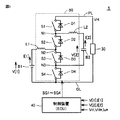•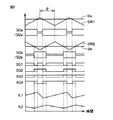•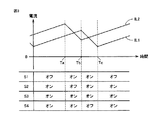••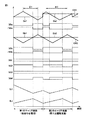•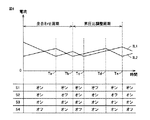•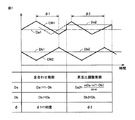•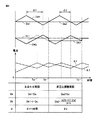•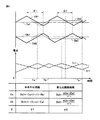•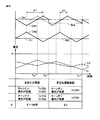•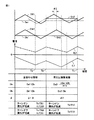•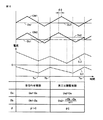•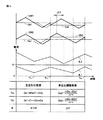•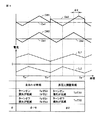•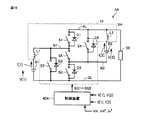•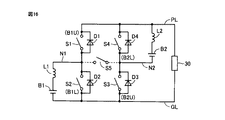•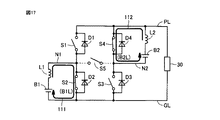•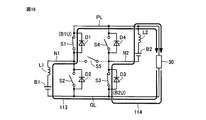•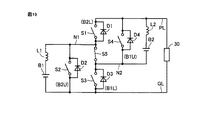•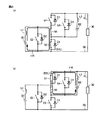•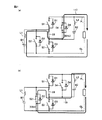•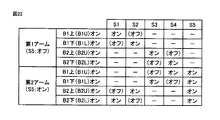••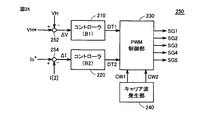••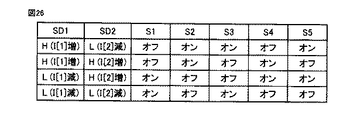•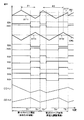•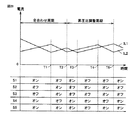•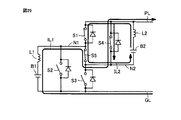•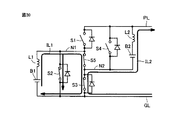•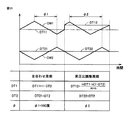## Description

The present invention relates to a power supply system, and more particularly to a power supply system for executing DC power conversion between two DC power supplies and a load.

A hybrid power supply system that uses a power converter connected between a plurality of power supplies and a load to supply power to the load by combining the plurality of power supplies is used.

For example, Japanese Patent Laying-Open No. 2013-13234 (Patent Document 1) describes a configuration of a power converter that can switch an operation mode by switching switching patterns of a plurality of switching elements. The operation mode includes a mode (parallel connection mode) in which DC power conversion is performed in a state where two DC power supplies are used in parallel.

JP2013-13234A

In the power converter described in Patent Document 1, DC power source conversion from two DC power sources is controlled by a plurality of switching elements sharing a current path in parallel connection mode. In Patent Document 1, a first carrier wave used for PWM (Pulse Width Modulation) control for controlling direct current power conversion from a first direct current power source and direct current power conversion from a second direct current power source are controlled. Therefore, the switching loss of the plurality of switching elements is reduced by adjusting the phase difference with the second carrier wave used for the PWM control. However, there is a limit to the effect of reducing the switching loss only by adjusting the phase difference between the first and second carrier waves.

The present invention has been made to solve such problems, and its object is to reduce the switching loss of a power supply system having two DC power supplies and to improve the efficiency of DC power conversion. That is.

In one aspect of the present invention, a power supply system for controlling a DC voltage between a first power line on a high voltage side and a second power line on a low voltage side includes a first DC power supply and a second DC power supply. A power converter for executing DC power conversion in parallel between the first and second DC power supplies and the first and second power lines, and a control for controlling DC voltage conversion in the power converter Equipment. In response to a control signal from the first reactor, the second reactor, and the control device, the power converter includes a first reactor between the first DC power source and the first and second power lines. And alternately forming a first current path for storing energy and a second current path for discharging energy of the first reactor, and a second DC power source, first and second power lines, A plurality of switching devices configured to alternately form a third current path for storing energy in the second reactor and a fourth current path for discharging energy of the second reactor during Element. Each of the plurality of switching elements is arranged to be included in both one of the first and second current paths and either one of the third and fourth current paths. The control device takes m carrier cycles (m is an integer of 2 or more) as one adjustment period, and sets the first output duty ratio from the first DC power source and the first in each carrier period within the one adjustment period. The first pulse width modulation control based on the comparison with the second carrier wave is executed, and the second pulse width modulation control based on the comparison between the second output duty ratio from the second DC power source and the second carrier wave is performed. Run. The plurality of switching elements are controlled so as to increase or decrease the current of the first reactor according to the first pulse width modulation control, and to control the increase or decrease of the current of the second reactor according to the second pulse width modulation control. , On and off according to the control signal. The m carrier periods are composed of n (n is an integer greater than or equal to 1 and less than m) first carrier periods and (mn) second carrier periods. In the first carrier cycle, the control device sets the first and second inflection points so that the two inflection points of the current of the first reactor and the two inflection points of the current of the second reactor have the same timing, respectively. The output duty ratio of 2 is adjusted, and the phase difference between the first carrier wave and the second carrier wave is adjusted. In the second carrier period, the control device adjusts the first and second output duty ratios based on the first and second output duty ratios in the first carrier period, and further, the current of the first reactor The phase difference is adjusted so that one of the two inflection points and one of the two inflection points of the current of the second reactor have the same timing.

Preferably, in the second carrier cycle, the control device is configured such that the average value of the first output duty ratio for one adjustment period corresponds to the duty ratio for controlling the output from the first DC power supply. 1st and 2nd in the 1st carrier cycle so that the average value of the 2nd output duty ratio which added up 1 adjustment period may correspond to the duty ratio for controlling the output from the 2nd direct-current power supply. The first and second output duty ratios are adjusted based on the output duty ratio.

Preferably, in the first carrier cycle, the control device determines whether the first DC power source is in a power running state or a regenerative state, and whether the second DC power source is in a power running state or a regenerative state. Based on the combination, the first and second output duty ratios are adjusted, and the phase difference between the first carrier wave and the second carrier wave is adjusted.

Preferably, when the first DC power source and the second DC power source are in a power running state, or when the first DC power source and the second DC power source are in a regenerative state, the control device includes: In the carrier cycle, the inflection point of the current of the first reactor when switching from the first current path to the second current path, and the second reactor when switching from the fourth current path to the third current path The inflection point of the current of the first reactor and the inflection point of the current of the first reactor when switching from the second current path to the first current path, and the fourth from the third current path. The first and second output duty ratios are adjusted and the phase difference is adjusted so that the inflection point of the current of the second reactor when switching to the current path is at the same timing.

Preferably, when the first DC power source and the second DC power source are in a power running state, or when the first DC power source and the second DC power source are in a regenerative state, the control device includes: In the carrier period, the first and second output duty ratios are adjusted and the phase difference is adjusted to 180 degrees so that the sum of the first output duty ratio and the second output duty ratio becomes 1.

Preferably, when the first DC power source is in a regenerative state and the second DC power source is in a power running state, or the first DC power source is in a power running state, and the second DC power source is in a regenerative state. When the state is in the state, the control device determines that the inflection point of the current of the first reactor when switching from the first current path to the second current path is the third current path in the first carrier cycle. Change of the current of the first reactor when switching from the second current path to the first current path, and at the same timing as the inflection point of the current of the second reactor when switching from the current path to the fourth current path. The first and second output duty ratios are adjusted so that the inflection point and the inflection point of the current of the second reactor when switching from the fourth current path to the third current path have the same timing. At the same time, the phase difference is adjusted.

Preferably, when the first DC power source is in a regenerative state and the second DC power source is in a power running state, or the first DC power source is in a power running state, and the second DC power source is in a regenerative state. In the state, the control device adjusts the first and second output duty ratios so that the first output duty ratio and the second output duty ratio are equal in the first carrier period. At the same time, the phase difference is adjusted to zero.

Preferably, the plurality of switching elements are electrically connected between the first switching element electrically connected between the first power line and the first node, and between the second node and the first node. And a third switching element electrically connected between the second node and the third node electrically connected to the negative terminal of the second DC power source And a fourth switching element electrically connected between the negative terminal of the first DC power supply and the third node. The first reactor is electrically connected in series with the first DC power source between the second node and the second power line. The second reactor is electrically connected in series with the second DC power source between the first node and the third notebook.

Preferably, the plurality of switching elements are electrically connected between the first power line and the first node, and between the second power line and the first node. A second switching element connected; a third switching element electrically connected between the second node and the second power line; and between the first power line and the second power node. A fourth switching element electrically connected; and a fifth switching element electrically connected between the first node and the second node. The first reactor is electrically connected in series with the first DC power source between the first node and the second power line. The second reactor is electrically connected in series with the second DC power source between the second node and the first power line.

According to the present invention, it is possible to reduce the switching loss of a power supply system including two DC power supplies and to improve the efficiency of DC power conversion.

It is a circuit diagram which shows the structural example of the power supply system according to Embodiment 1 of this invention. It is a wave form diagram which shows the control operation example of a parallel control mode in case the carrier wave from which a phase differs is used. It is a wave form diagram for demonstrating the operation example of the carrier phase control in parallel connection mode. FIG. 4 is a circuit diagram illustrating a current path in a predetermined period of FIG. 3. It is a wave form diagram for demonstrating application of duty ratio control with respect to the power converter according to Embodiment 1. FIG. It is a wave form diagram explaining the current phase by duty ratio control and carrier phase control by Embodiment 1 for reducing switching loss in parallel connection mode. 5 is a chart for illustrating duty ratio control according to the first embodiment. 12 is a chart for illustrating a first modification of duty ratio control according to the first embodiment. 12 is a chart for illustrating a second modification of duty ratio control according to the first embodiment. 6 is a chart for explaining duty ratio control and carrier phase control according to the first embodiment when both DC power supplies B1 and B2 are in a regenerative state. 5 is a chart for explaining duty ratio control and carrier phase control according to Embodiment 1 when DC power supply B1 is in a regenerative state and DC power supply B2 is in a powering state. 11 is a chart for illustrating a third modification of duty ratio control according to the first embodiment. 10 is a diagram for illustrating a fourth modification of duty ratio control according to the first embodiment. 6 is a chart for explaining duty ratio control and carrier phase control according to the first embodiment when DC power supply B1 is in a power running state and DC power supply B2 is in a regenerative state. It is a circuit diagram which shows the structural example of the power supply system according to Embodiment 2 of this invention. FIG. 3 is a first equivalent circuit diagram of the power converter shown in FIG. 1 in a parallel boost mode. FIG. 17 is a circuit diagram showing a current path when the lower arm of each DC power supply is turned on in the equivalent circuit diagram shown in FIG. 16. FIG. 17 is a circuit diagram showing a current path when the upper arm of each DC power supply is on in the equivalent circuit diagram shown in FIG. 16. FIG. 6 is a second equivalent circuit diagram in the parallel boost mode of the power converter shown in FIG. 1. FIG. 20 is a circuit diagram showing a current path when the lower arm of each DC power supply in the equivalent circuit diagram shown in FIG. 19 is on. FIG. 20 is a circuit diagram showing a current path when the upper arm of each DC power supply is on in the equivalent circuit diagram shown in FIG. 19. It is a graph which shows the correspondence of ON / OFF of each arm of the step-up chopper circuit using a 1st arm and a 2nd arm, and ON / OFF of a switching element. 5 is a chart showing a list of logical operation expressions for controlling on / off of each switching element in a parallel boost mode. It is a functional block diagram for demonstrating the output control example of the DC power supply in the parallel boost mode of the power converter shown in FIG. FIG. 25 is a waveform diagram for explaining the operation of the PWM control unit shown in FIG. 24. 6 is a chart showing a list of switching patterns in a parallel boost mode. FIG. 11 is a waveform diagram for explaining application of duty ratio control to a power converter according to a second embodiment. It is a wave form diagram explaining the current phase by duty ratio control and carrier phase control by Embodiment 2 for reducing switching loss in parallel boost mode. FIG. 29 is a circuit diagram illustrating a current path in a predetermined period of FIG. FIG. 29 is a circuit diagram illustrating a current path in a predetermined period of FIG. 12 is a chart for illustrating duty ratio control according to the second embodiment.

Hereinafter, embodiments of the present invention will be described in detail with reference to the drawings. Hereinafter, a plurality of embodiments will be described. However, it is planned from the beginning of the application to appropriately combine the configurations described in the embodiments. In the drawings, the same or corresponding parts are denoted by the same reference numerals and description thereof will not be repeated.

[Embodiment 1]
(Circuit configuration)
FIG. 1 is a circuit diagram showing a configuration example of a power supply system according to the first embodiment of the present invention. The power supply system 5 according to the first embodiment has the same circuit configuration as that of the power supply system disclosed in Patent Document 1.

Referring to FIG. 1, power supply system 5 includes a DC power supply B <b> 1, a DC power supply B <b> 2, a load 30, a control device 40, and a power converter 50.

In the first embodiment, DC power supplies B1 and B2 are configured by a power storage device such as a secondary battery or an electric double layer capacitor. For example, DC power supply B1 is comprised with secondary batteries, such as a lithium ion secondary battery and a nickel metal hydride battery. The DC power source B2 is constituted by a DC voltage source element having excellent output characteristics such as an electric double layer capacitor and a lithium ion capacitor. The DC power supply B1 and the DC power supply B2 correspond to “first DC power supply” and “second DC power supply”, respectively.

Note that the DC power supplies B1 and B2 can be configured by the same type of power storage device. Further, the capacities of the DC power supplies B1 and B2 are not particularly limited, and each of the DC power supplies B1 and B2 may be configured with an equivalent capacity, and the capacity of one DC power supply may be the same as that of the other DC power supply. It may be larger than the capacity.

The power converter 50 is configured to control a DC voltage VH (hereinafter also referred to as an output voltage VH) between the high voltage side power line PL and the low voltage side power line GL. The power line GL is typically constituted by a ground wiring.

The load 30 operates in response to the output voltage VH of the power converter 50. Voltage command value VH * of output voltage VH is set to a voltage suitable for the operation of load 30. Voltage command value VH * may be variably set according to the state of load 30. Furthermore, the load 30 may be configured to be able to generate charging power for the DC power sources B1 and / or B2 by regenerative power generation or the like.

Power converter 50 includes power semiconductor switching elements S1 to S4 and reactors L1 and L2. In the first embodiment, power semiconductor switching elements (hereinafter also simply referred to as “switching elements”) include IGBTs (Insulated Gate Bipolar Transistors), power MOS (Metal Oxide Semiconductor) transistors, or power bipolar transistors. Can be used. Anti-parallel diodes D1 to D4 are arranged for switching elements S1 to S4. The switching elements S1 to S4 can be turned on and off in response to control signals SG1 to SG4 from the control device 40.

Switching element S1 is electrically connected between power line PL and node N1. Reactor L2 and DC power supply B2 are electrically connected in series between nodes N1 and N3. For example, reactor L2 is electrically connected between node N1 and the positive terminal of DC power supply B2, and the negative terminal of DC power supply B2 is electrically connected to node N3. Even if the connection order of the reactor L2 and the DC power supply B2 is changed, an electrically equivalent circuit configuration is formed.

Switching element S2 is electrically connected between nodes N1 and N2. Reactor L1 and DC power supply B1 are electrically connected in series between node N2 and power line GL. For example, reactor L1 is electrically connected between the positive terminal of DC power supply B1 and node N1, and the negative terminal of DC power supply B1 is electrically connected to power line GL. Even if the connection order of the reactor L1 and the DC power supply B1 is changed, an electrically equivalent circuit configuration is formed.

Switching element S3 is electrically connected between nodes N2 and N3. Switching element S4 is electrically connected between node N3 and power line GL. Power line GL is electrically connected to load 30 and the negative terminal of DC power supply B1.

In the configuration example of FIG. 1, the switching element S1 corresponds to a “first switching element”, the switching element S2 corresponds to a “second switching element”, and the switching element S3 corresponds to a “third switching element”. The switching element S4 corresponds to a “fourth switching element”. Furthermore, reactors L1 and L2 correspond to a “first reactor” and a “second reactor”, respectively.

The control device 40 is configured by, for example, an electronic control unit (ECU) having a CPU (Central Processing Unit) and a memory (not shown). The control device 40 is configured to perform arithmetic processing using the detection values of each sensor based on the map and program stored in the memory. Alternatively, at least a part of the control device 40 may be configured to execute predetermined numerical / logical operation processing by hardware such as an electronic circuit.

The control device 40 generates control signals SG1 to SG4 for controlling on / off of the switching elements S1 to S4 in order to control the output voltage VH.

Although not shown in FIG. 1, the voltage (denoted as V ) and current (denoted as I ) of the DC power supply B1, and the voltage (V  of the DC power supply B2). And a detector (voltage sensor, current sensor) for output voltage VH and current (denoted as I ) and output voltage VH. The outputs of these detectors are provided to the controller 40.

Power converter 50 according to the first embodiment is connected in series with a “parallel connection mode” in which DC power supplies B1 and B2 transfer power to and from load 30 in parallel under the control of switching elements S1 to S4. The DC power supplies B1 and B2 can be operated by switching the “series connection mode” in which power is exchanged with the load 30. In the first embodiment, a control operation in the parallel connection mode, particularly control for reducing power loss by the switching element will be described.

(Circuit operation in parallel connection mode)
A control operation in the parallel connection mode of the power converter 50 will be described.

Referring to FIG. 1, power converter 50 is configured to include a boost chopper circuit corresponding to each of DC power supply B1 and DC power supply B2. That is, for DC power supply B1, a current bidirectional first step-up chopper circuit is configured with switching elements S1 and S2 as upper arms and switching elements S3 and S4 as lower arms.

Specifically, the lower arm (switching elements S3 and S4) of the first step-up chopper circuit forms a “first current path” for storing energy in reactor L1 when DC power supply B1 is in a powering state. On the other hand, when the DC power supply B1 is in the regenerative state, a “second current path” is formed for outputting the stored energy of the reactor L1 to the DC power supply B1. The upper arms (switching elements S1 and S2) of the first step-up chopper circuit “second” for outputting the stored energy of the reactor L1 to the power line PL together with the energy of the DC power supply B1 when the DC power supply B1 is in the power running state. While the “current path” is formed, a “first current path” for storing energy in the reactor L1 from the output from the power line PL when the DC power supply B1 is in the regenerative state is formed.

While the pair of switching elements S3 and S4 is turned on, the first period in which at least one of the switching elements S1 and S2 is turned off and the pair of switching elements S1 and S2 are turned on, while the switching element S3 , S4 and the second period in which at least one of them is turned off alternately, the first current path and the second current path are alternately formed.

In the DC power conversion for the DC power supply B1 in such a parallel connection mode, the voltage conversion ratio (step-up ratio) is the voltage V  of the DC power supply B1, the output voltage VH of the power line PL, and the output duty ratio Da of the DC power supply B1. (Hereinafter, also simply referred to as a duty ratio Da) is expressed by the following equation (1). The duty ratio is defined as the ratio of the lower arm on period to the switching period, which is the sum of the lower arm on period and off period. In the equation (1), the duty ratio in the first period when the pair of switching elements S3 and S4 is turned on is Da.

VH = 1 / (1-Da) · V  (1)
Similarly, for the DC power source B2, a current bidirectional second step-up chopper circuit is configured with the switching elements S1 and S4 as upper arms and the switching elements S2 and S3 as lower arms. The lower arms (switching elements S2, S3) of the second boost chopper circuit form a “third current path” for storing energy in the reactor L2 when the DC power supply B2 is in a power running state, A “fourth current path” is formed for outputting the energy stored in reactor L2 to DC power supply B2 when power supply B2 is in the regenerative state. The upper arm (switching elements S1 and S4) of the second boost chopper circuit outputs “the fourth energy stored in reactor L2 together with the energy of DC power supply B2 to power line PL when DC power supply B2 is in a powering state. While the “current path” is formed, a “third current path” is formed for accumulating the energy from the output from the power line PL in the reactor L2 when the DC power supply B2 is in the regenerative state.

While the pair of switching elements S2 and S3 is turned on, the first period when at least one of the switching elements S1 and S4 is turned off, and the pair of switching elements S1 and S4 is turned on, while the switching element S2 , S3 and the second period in which at least one of them is turned off alternately, the third current path and the fourth current path are alternately formed.

In the DC power conversion for the DC power supply B2 in such a parallel connection mode, the voltage conversion ratio (step-up ratio) is the voltage V  of the DC power supply B2, the output voltage VH of the power line PL, and the output duty ratio from the DC power supply B2. Using Db (hereinafter, also simply referred to as duty ratio Db), the following equation (2) is used. In the equation (2), Db is a duty ratio in a first period in which the pair of switching elements S2 and S3 is turned on.

VH = 1 / (1-Db) · V  (2)
As can be understood from FIG. 1, each of the switching elements S1 to S4 is one of the first and second current paths formed between the DC power supply B1 and the power line PL by the first boost chopper circuit. One of them is arranged so as to be included in both one of the third and fourth current paths formed between DC power supply B2 and power line PL by the second boost chopper circuit.

(Control operation in parallel connection mode)
A control operation in the parallel connection mode in the power converter 50 will be described. The control operation described below is realized by hardware processing and / or software processing by the control device 40.

When power converter 50 is operated in the parallel connection mode, pulse width modulation (PWM) control is executed in parallel for each of DC power supply B1 and DC power supply B2. In the parallel connection mode, as in the parallel connection mode described in Patent Document 1, one output of the DC power supplies B1 and B2 is controlled to compensate for the voltage deviation ΔV (ΔV = VH * −VH) of the output voltage VH. In addition to (voltage control), the other output of the DC power supplies B1 and B2 can be controlled (current control) so as to compensate for the current deviation of the current I  or I . For example, the current control command value (Io *) can be set to control the output power of the power source.

As an example, the duty ratio Da of the DC power supply B1 is calculated by voltage feedback control for operating the DC power supply B1 as a voltage source or current feedback control for operating as a current source. The duty ratio Db of the DC power supply B2 is calculated by current feedback control for operating the DC power supply B2 as a current source when the DC power supply B1 operates as a voltage source. On the contrary, the duty ratio Db is calculated by voltage feedback control for operating the DC power supply B2 as a voltage source when the DC power supply B1 operates as a current source.

(Control operation to reduce switching loss in parallel connection mode)
Since the basic control operation in the parallel connection mode of the power converter 50 is equivalent to the control operation in the parallel connection mode of Patent Document 1, detailed description thereof will be omitted. Here, carrier wave phase control (hereinafter also referred to as “carrier phase control”) used for PWM control of DC power supply B1 and DC power supply B2 will be described.

(1) Carrier Phase Control FIG. 2 is a waveform diagram showing a control operation example in the parallel control mode when carrier waves having different phases are used.

Referring to FIG. 2, carrier wave CW1 used for PWM control of DC power supply B1 and carrier wave CW2 used for PWM control of DC power supply B2 have the same frequency but different phases. In the example of FIG. 2, the phase difference between carrier waves CW1 and CW2 is φ = 180 degrees. Triangular waves are used for the carrier waves CW1 and CW2. The period of carrier waves CW1 and CW2 corresponds to the switching frequency of each switching element. The amplitude of the carrier wave CW1 is set to a voltage corresponding to the duty ratio Da = 1.0. The amplitude of carrier wave CW2 is set to a voltage corresponding to duty ratio Db = 1.0.

A control pulse signal SDa is generated based on a voltage comparison between the duty ratio Da for controlling (voltage or current control) the output of the DC power supply B1 and the carrier wave CW1. Control pulse signal SDa is set to a logic high level (hereinafter referred to as H level) when the voltage indicating duty ratio Da is higher than the voltage of carrier wave CW1, while it is lower than the voltage of carrier wave CW1. It is set to a logic low level (hereinafter referred to as L level). The ratio of the H level period to the cycle (H level period + L level period) of the control pulse signal SDa, that is, the duty ratio of the control pulse signal SDa is equal to Da.

Control pulse signal / SDa is an inverted signal of control pulse signal SDa. As the duty ratio Da increases, the H level period of the control pulse signal SDa increases. On the other hand, when the duty ratio Da decreases, the L level period of the control pulse signal SDa increases.

The control pulse signal SDa corresponds to a signal for controlling on / off of the lower arms (switching elements S3 and S4) of the first boost chopper circuit described above. On the other hand, the control pulse signal / SDa corresponds to a signal for controlling on / off of the upper arms (switching elements S1, S2) of the first boost chopper circuit.

Similarly, control pulse signal SDb and its inverted signal / SDb are generated based on voltage comparison between duty ratio Db for controlling output (current or voltage control) of DC power supply B2 and carrier wave CW2. . The duty ratio of control pulse signal SDb is the same as Db, and the duty ratio of control pulse signal / SDb is equivalent to (1.0−Db). That is, as the duty ratio Db increases, the H level period of the control pulse signal SDb increases. On the contrary, when the duty ratio Db decreases, the L level period of the control pulse signal SDb increases.

The control pulse signal SDb corresponds to a signal for controlling on / off of the lower arms (switching elements S2, S3) of the second boost chopper circuit described above. On the other hand, control pulse signal / SDb corresponds to a signal for controlling on / off of the upper arm (switching elements S1, S4) of the second boost chopper circuit.

Control signals SG1 to SG4 are set based on a logical operation of control pulse signals SDa (/ SDa) and SDb (/ SDb). By turning on and off switching elements S1 to S4 based on control signals SG1 to SG4, increase / decrease in reactor current IL1 flowing through reactor L1 and increase / decrease in reactor current IL2 flowing through reactor L2 are controlled as shown in FIG. Reactor current IL1 corresponds to current I  of DC power supply B1, and reactor current IL2 corresponds to current I  of DC power supply B2.

As shown in FIG. 2, by providing a phase difference φ between the carrier wave CW1 and the carrier wave CW2, the control pulse signal SDa is compared with the control pulse signal SDa when the phase difference φ = 0. Although the length of the H level period is the same, the phase is different. Similarly, control pulse signal SDb has the same H-level period length but a different phase compared to control pulse signal SDb when phase difference φ = 0. As a result, the control signals SG1 to SG4 have different waveforms from the control signals SG1 to SG4 when the phase difference φ = 0. Therefore, it is understood that the phase relationship (current phase) between reactor current IL1 and reactor current IL2 also changes by changing phase difference φ between carrier waves CW1 and CW2.

On the other hand, with respect to the same duty ratio Da, Db, it is understood that the average value of reactor currents IL1, IL2 does not change even when phase difference φ is changed. That is, the outputs of the DC power supplies B1 and B2 are controlled by the duty ratios Da and Db, and there is no effect even if the phase difference φ between the carrier waves CW1 and CW2 is changed.

Therefore, the power loss of switching elements S1 to S4 in the parallel connection mode of power converter 50 can be reduced by carrier phase control that appropriately adjusts phase difference φ between carrier waves CW1 and CW2.

In the following, as a representative example, control in a state where both DC power sources B1 and B2 are in a power running state, that is, a reactor current IL1> 0 and a reactor current IL2> 0 will be described.

FIG. 3 is a waveform diagram for explaining an operation example of carrier phase control in the parallel connection mode.

Referring to FIG. 3, switching elements S2 to S4 are turned on until time Ta, so that the lower arm (first and third current paths) of the boost chopper circuit with respect to both DC power supplies B1 and B2. Is turned on. For this reason, both reactor currents IL1 and IL2 rise.

At time Ta, the switching element S2 is turned off, so that the lower arm (third current path) of the step-up chopper circuit is turned off with respect to the DC power supply B2, so that the reactor current IL2 decreases from rising to falling. Turn. That is, reactor current IL2 becomes maximum. Instead of switching off the switching element S2, the switching element S1 is turned on.

After the time Ta, the lower arm (first current path) of the boost chopper circuit is turned on with respect to the DC power supply B1, and the lower arm of the boost chopper circuit is turned off with respect to the DC power supply B2. That is, reactor current IL2 rises, while reactor current IL1 falls. At this time, the current path in the power converter 50 is as shown in FIG.

As understood from FIG. 4A, after the time Ta, the difference current between the reactor currents IL1 and IL2 passes through the switching element S4. That is, the passing current of the switching element S4 becomes small.

Referring to FIG. 3 again, when switching element S4 is turned off from the state after time Tb, the lower arm of step-up chopper circuit is turned off with respect to DC power supply B1, so that reactor current IL1 falls from the rise. Turn to. That is, reactor current IL1 becomes maximum. Further, when switching element S2 is turned on, the lower arm of the step-up chopper circuit is turned on with respect to DC power supply B2, so that reactor current IL2 changes from falling to rising again. That is, reactor current IL2 is minimal. As a result, the current path in the power converter 50 changes from the state of FIG. 4A to the state of FIG. In the state of FIG. 4B, since the difference current between reactor currents IL1 and IL2 passes through switching element S2, the passing current of switching element S2 becomes small.

When the switching element S4 is turned off in the state of FIG. 4A, the current when the switching element S4 is turned off is reduced to perform soft switching, and the switching loss can be reduced. Further, by turning on the switching element S2 in the state of FIG. 4B, the current when the switching element S2 is turned on is reduced to perform soft switching, and the switching loss can be reduced.

Therefore, as shown in FIG. 3, the current phase, that is, the level of the carrier waves CW1 and CW2, is such that the timing at which the reactor current IL1 changes from rising to falling overlaps the timing at which the reactor current IL2 changes from falling to rising. Adjust the phase difference φ. That is, the phase difference φ is adjusted so that the inflection point (maximum point) of reactor current IL1 and the inflection point (minimum point) of reactor current IL2 are at the same timing. Thereby, at time Tb in FIG. 3, the switching element S2 is turned on and the switching element S4 is turned off.

Referring to FIG. 3 again, at time Tc, switching element S1 is turned off and switching element S4 is turned on. As a result, the lower arm of the step-up chopper circuit is turned off with respect to the DC power source B1, and the reactor current IL1 changes from falling to rising. That is, reactor current IL2 is minimal. When the switching element S1 is turned on instead of turning off the switching element S2, the lower arm of the step-up chopper circuit is turned on for each of the DC power supplies B1 and B2. Therefore, the state before time Ta described above is reproduced, and both reactor currents IL1 and IL2 rise.

Thus, when both DC power supplies B1 and B2 are in a power running state, the current phase is such that the maximum point of reactor current IL1 and the minimum point of reactor current IL2 overlap at time Tb in the figure. The phase difference φ between the carrier waves CW1 and CW2 is adjusted. Thereby, the turn-on loss of switching element S2 and the turn-off loss of switching element S4 at time Tb can be reduced.

However, as understood from FIG. 3, the inflection point (maximum point or minimum point) of reactor current IL1 and the inflection point (maximum point or minimum point) of reactor current IL2 are adjusted by adjusting phase difference φ. The coincidence timing is only once in one period (hereinafter also referred to as one carrier period) of the carrier waves CW1 and CW2. In other words, the timing at which switching loss can be reduced by carrier phase control is only once in one carrier period.

Therefore, at time Ta, which is the timing when the reactor current IL2 becomes maximum, the current at the time of turn-off of the switching element S2 is large and hard switching is performed, so there is a concern that the turn-off loss of the switching element S2 increases. Similarly, at the time Tc when the reactor current IL1 is minimized, the current at the turn-on of the switching element S4 is large and hard switching is performed, so there is a concern that the turn-on loss of the switching element S4 increases.

In contrast, in the parallel connection mode of power converter 50 according to the first embodiment, as will be described below, in parallel with carrier phase control, duty ratio Da of DC power supply B1 and duty ratio Db of DC power supply B2 are set. By performing control to appropriately adjust (hereinafter also referred to as “duty ratio control”), the switching loss of the switching elements S1 to S4 is further reduced.

(2) Duty Ratio Control FIG. 5 is a waveform diagram for explaining the application of duty ratio control to power converter 50 according to the first embodiment.

Referring to FIG. 5, when duty ratio control is applied, by adjusting duty ratios Da and Db, H level period of control pulse signal SDa (= control pulse signal / L level period of SDa) and control pulse signal SDb H level period (= control pulse signal / L level period of SDb) is adjusted.

As described above, the carrier phase control changes the phase relationship (current phase) between the reactor current IL1 and the reactor current IL2 by changing the phase difference φ between the carrier waves CW1 and CW2. On the other hand, in the duty ratio control, the waveforms of the reactor currents IL1 and IL2 are changed by changing the duty ratios Da and Db. In the first embodiment, by combining carrier phase control and duty ratio control, the timing at which the inflection point of reactor current IL1 and the inflection point of reactor current IL2 coincide with each other in one carrier cycle can be increased.

On the other hand, when the duty ratios Da and Db are changed, the outputs of the DC power supplies B1 and B2 are also changed. As a result, the power distribution between the DC power supplies B1 and B2 changes, and it becomes difficult to control the output voltage VH to the voltage command value VH *.

Therefore, in power converter 50 according to the first embodiment, the average value of duty ratio Da obtained by adding a plurality of carrier periods to “one adjustment period” controls the output of DC power supply B1 (voltage / voltage). Current ratio), and the average value of the duty ratio Db for one adjustment period corresponds to the duty ratio for controlling (current / voltage control) the output of the DC power supply B2. The duty ratios Da and Db in each carrier cycle are adjusted. That is, the duty ratio for output control of the DC power supplies B1 and B2 is ensured in units of one adjustment period. Thus, even if the duty ratios Da and Db in each carrier cycle are changed, the power distribution between the DC power sources B1 and B2 is not affected.

Specifically, m carrier cycles (m is an integer equal to or greater than 2) are defined as one adjustment period, and n (n is an integer equal to or greater than 1 and less than m) “first carrier cycle”. And (mn) “second carrier periods”. FIG. 5 shows an operation example of the power converter 50 when m = 2 and n = 1 for simplification of description. That is, FIG. 5 shows an example in which the first carrier period and the second carrier period are alternately formed.

In the first carrier cycle, the duty ratios Da and Db are set so that the two inflection points (maximum point and minimum point) of the reactor current IL1 and the two inflection points of the reactor current IL2 have the same timing. While adjusting, the phase difference φ is adjusted. In FIG. 5, the duty ratio of the DC power supply B1 in the first carrier cycle is Da1, and the duty ratio of the DC power supply B2 is Db1. Further, the phase difference in the first carrier period is φ1.

On the other hand, in the second carrier period, the duty ratios Da and Db are adjusted based on the duty ratios Da1 and Db2 in the first carrier period. Specifically, the duty ratio in the first carrier cycle is such that the average values of the duty ratios Da and Db for one adjustment period correspond to the duty ratio calculated by the output control of the DC power supplies B1 and B2, respectively. The duty ratios Da and Db are adjusted based on Da1 and Db2. In FIG. 5, the duty ratio of the DC power supply B1 in the second carrier cycle is Da2, and the duty ratio of the DC power supply B2 is Db2. Further, the duty ratio Da2, adjusted so that one of the two inflection points (maximum point) of the reactor current IL1 and one of the two inflection points (minimum point) of the reactor current IL2 are at the same timing. The phase difference φ is adjusted according to Db2. The phase difference in the second carrier period is φ2.

That is, the first carrier period corresponds to a period (hereinafter, also referred to as “total alignment period”) in which control specialized in matching the timings of the two inflection points of reactor currents IL1 and IL2 is performed. On the other hand, the second carrier cycle is a cycle in which control for ensuring the duty ratio for output control of the DC power supplies B1 and B2 is performed in units of one adjustment period (hereinafter referred to as “step-up ratio adjustment cycle”). It corresponds to). Also in the step-up ratio adjustment cycle, the phase difference φ is adjusted so that the inflection points of reactor currents IL1 and IL2 have the same timing.

Next, the operation and control of power converter 50 in the first carrier period and the second carrier period will be described in detail. In the following, as a representative example, control in a state where both DC power sources B1 and B2 are in a power running state, that is, a reactor current IL1> 0 and a reactor current IL2> 0 will be described.

FIG. 6 is a waveform diagram illustrating the current phase by duty ratio control and carrier phase control according to the first embodiment for reducing switching loss in the parallel connection mode in power converter 50.

(2-1) Control Operation in First Carrier Cycle Referring to FIG. 6, switching elements S1 and S2 are turned on until time Ta, so that the upper arm (the first step of the boost chopper circuit with respect to DC power supply B1) 2 current path) is turned on. For this reason, reactor current IL1 falls. Further, since the switching elements S2 and S3 are turned on, the lower arm (third current path) of the boost chopper circuit is turned on with respect to the DC power supply B2. For this reason, reactor current IL2 rises. That is, reactor current IL1 decreases while reactor current IL2 increases. At this time, the current path in the power converter 50 is as shown in FIG. In the state of FIG. 4B, since the difference current between reactor currents IL1 and IL2 passes through switching element S2, the passing current of switching element S2 becomes small.

At time Ta, switching element S4 is turned on, so that lower arm (first current path) of boost chopper circuit is turned on with respect to DC power supply B1, and reactor current IL1 starts to rise. . When switching element S2 is turned off, the upper arm (fourth current path) of the step-up chopper circuit is turned off with respect to DC power supply B2, so that reactor current IL2 starts to fall. That is, the current path in the power converter 50 changes from the state shown in FIG. 4B to the state shown in FIG. In the state of FIG. 4A, since the difference current between reactor currents IL1 and IL2 passes through switching element S4, the passing current of switching element S4 becomes small.

By turning off the switching element S2 in the state of FIG. 4B, the current at the time of turning off the switching element S2, that is, the turn-off loss can be reduced. Further, by turning on the switching element S4 in the state of FIG. 4A, it is possible to reduce the current when the switching element S4 is turned on, that is, the turn-on loss.

Therefore, as shown in FIG. 6, the duty ratios Da1 and Db1 are adjusted and the phase difference φ1 is adjusted so that the rising timing of the reactor current IL1 and the falling timing of the reactor current IL2 overlap. Thereby, at time Ta, switching element S2 is turned off and switching element S4 is turned on. The rising timing of reactor current IL1 corresponds to the inflection point of reactor current IL1 when switching from the second current path to the first current path, and the falling timing of reactor current IL2 is from the third current path. This corresponds to the inflection point of the reactor current IL2 when switching to the fourth current path.

Further, in the first embodiment, switching element S2 is turned on and switching element S4 is turned off at time Tb, similarly to time Tb in FIG. Thereby, reactor current IL1 starts to fall again, and reactor current IL2 starts to rise again. That is, the current path in the power converter 50 changes from the state shown in FIG. 4A to the state shown in FIG. Since the falling timing of reactor current IL1 and the rising timing of reactor current IL2 coincide at time Tb, the turn-on loss of switching element S2 and the turn-off loss of switching element S4 at time Tb can be reduced. The fall timing of reactor current IL1 corresponds to the inflection point of reactor current IL1 when switching from the first current path to the second current path, and the rise timing of reactor current IL2 is from the fourth current path. This corresponds to the inflection point of the reactor current IL2 when switching to the third current path.

As described above, in the first carrier cycle, the duty ratios Da1 and Db1 are adjusted and the phase difference φ between the carrier waves CW1 and CW2 is adjusted so that both of the DC power sources B1 and B2 are in a power running state. The rise timing of reactor current IL1 and the fall timing of reactor current IL2 overlap, and the fall timing of reactor current IL1 and the rise timing of reactor current IL2 overlap. That is, the turn-off timing of the switching element S2 matches the turn-on timing of the switching element S4, and the turn-on timing of the switching element S2 matches the turn-off timing of the switching element S4. Thus, in the first carrier cycle, the two inflection points of the reactor current IL1 and the two inflection points of the reactor current IL2 are respectively at the same timing, so that the carrier phase control shown in FIG. In comparison, it is understood that the switching loss in the switching elements S1 to S4 is further reduced.

(2-2) Control operation in second carrier period In the second carrier period from time Tb to Te, the average of the duty ratio Da obtained by adding the first carrier period and the second carrier period (one adjustment period) The duty ratio Da2 is adjusted so that the value corresponds to the duty ratio Da for controlling the output (voltage / current control) of the DC power supply B1. Similarly, the average value of the duty ratio Db obtained by adding the first carrier period and the second carrier period (one adjustment period) becomes the duty ratio Db for controlling (current / voltage control) the output of the DC power supply B2. The duty ratio Db2 is adjusted so as to correspond.

At time Tc, the switching element S1 is turned off and the switching element S4 is turned on. As a result, the lower arm of the step-up chopper circuit is turned on for each of the DC power supplies B1 and B2. As a result, both reactor currents IL1 and IL2 rise.

At time Td, switching element S1 is turned on and switching element S2 is turned off, so that the upper arm of the boost chopper circuit is turned on with respect to DC power supply B2, and reactor current IL2 decreases. Start. Thereby, the state after the time Ta is reproduced. In the second carrier period, similarly to the above-described time Tb, the phase difference φ2 is adjusted so that the falling timing of the reactor current IL1 and the rising timing of the reactor current IL2 overlap. Thereby, at the time Te, the switching element S2 is turned on and the switching element S4 is turned off. Therefore, in the second carrier cycle, the turn-on loss of switching element S2 and the turn-off loss of switching element S4 at time Te can be reduced.

FIG. 7 is a table for describing duty ratio control according to the first embodiment. FIG. 7 shows the setting of the duty ratios Da1 and Da2 when the duty ratios Db1 and Db2 are set to the duty ratio Db for output control of the DC power supply B2 (Db1 = Db2 = Db).

Referring to FIG. 7, in the first carrier period (all matching periods), duty ratio Da1 is adjusted so that the sum of duty ratio Da1 and duty ratio Db1 is 1 (Da1 + Db1 = 1). The duty ratio Da1 is set according to the duty ratio Db1 (= Db) as shown in the following equation (3).

Da1 = 1−Db1 = 1−Db (3)
In the second carrier cycle (step-up ratio adjustment cycle), the duty ratio Da2 is an average value of the duty ratio Da obtained by adding one adjustment period (m carrier cycles), and the duty ratio for output control of the DC power supply B1. It is set according to the duty ratio Da1 so as to correspond to Da. The duty ratio Da2 is set according to the duty ratio Da1, as shown in the following equation (4).

Da2 = (mDa-nDa1) / (mn) = {mDa-n (1-Db)} / (mn) (4)
In the operation example (m = 2, n = 1) shown in FIG. 5, the duty ratio Da2 is set to the duty ratio Da2 = 2 × Da− (1−Db) based on the equation (4). .

Here, as understood from FIG. 7, the phase difference φ1 = 180 degrees in the first carrier period. On the other hand, the phase difference φ2 in the second carrier period changes according to the duty ratios Da2 and Db2, that is, the duty ratios Da and Db. For this reason, the relationship between the duty ratios Da and Db and the phase difference φ2 is obtained in advance, and the corresponding relationship is previously determined as a map (hereinafter also referred to as “phase difference map”) or a relational expression (hereinafter referred to as “phase difference calculation formula”). Can be stored in the control device 40.

Then, in the PWM control for voltage / current control in the DC power supplies B1 and B2 in the parallel connection mode described with reference to FIG. 5, based on the calculated duty ratios Da and Db, the above formula (3) and formula The duty ratios Da1 and Da2 are set using (4), and the duty ratios Db1 and Db2 are set. Further, the phase difference φ2 can be calculated based on the duty ratios Da and Db according to the phase difference map and the phase difference calculation formula, while setting the phase difference φ1 = 180 degrees in the first carrier period. Then, in one adjustment period unit, the set phase differences φ1 and φ2 are switched to generate carrier waves CW1 and CW2, and PWM control based on the comparison between the duty ratio Da1 or Da2 and the carrier wave CW1, and the duty ratio Db1 or PWM control based on a comparison between Db2 and carrier wave CW2 is executed. In this way, high-efficiency DC power conversion with reduced switching loss in the switching elements S1 to S4 described above can be realized.

In FIGS. 5 to 7, the duty ratios Db1 and Db2 are set to the duty ratio Db calculated by the output control of the DC power supply B2, and the duty ratios Da1 and Da2 are adjusted. However, the duty ratio Da1, When Da2 is set to the duty ratio Da for output control of the DC power supply B1 and the duty ratios Db1 and Db2 are adjusted (first modification), or the duty ratios Da1 and Da2 and Db1 and Db2 are adjusted respectively. In the case (second modification), the same duty ratio control and carrier phase control can be executed.

(First modification)
FIG. 8 is a table for illustrating a first modification of the duty ratio control according to the first embodiment. FIG. 8 shows the setting of the duty ratios Db1 and Db2 when the duty ratios Da1 and Da2 are set to the duty ratio Da for output control of the DC power supply B1 (Da1 = Da2 = Da).

Referring to FIG. 8, duty ratio Db1 is adjusted so that the sum of duty ratio Da1 and duty ratio Db1 becomes 1 in the first carrier period (all matching periods). The duty ratio Db1 is set according to the duty ratio Da1 (= Da) as shown in the following equation (5).

Db1 = 1-Da1 = 1-Da (5)
In the second carrier period (step-up ratio adjustment period), the duty ratio Db2 is an average value of the duty ratio Db obtained by adding one adjustment period (m carrier periods), and the duty ratio for output control of the DC power supply B2 It is set according to the duty ratio Db1 so as to coincide with Db. The duty ratio Db2 is set according to the duty ratio Db1, as shown in the following equation (6).

Db2 = (mDb-nDb1) / (mn) = {mDb-n (1-Da)} / (mn) (6)
In the operation example (m = 2, n = 1) shown in FIG. 8, the duty ratio Db2 is set to the duty ratio Db2 = 2 × Db− (1−Da) based on the equation (6).

As can be understood from FIG. 8, the phase difference φ1 = 180 degrees in the first carrier period. On the other hand, the phase difference φ2 in the second carrier period is calculated based on the duty ratios Da and Db according to the phase difference map and the phase difference calculation formula.

(Second modification)
FIG. 9 is a table for describing a second modification of the duty ratio control according to the first embodiment.

Referring to FIG. 9, duty ratio Da1 and duty ratio Db1 are adjusted so that the sum of duty ratio Da1 and duty ratio Db1 becomes 1 in the first carrier period (all matching periods). When the duty ratio Da1 is set to the duty ratio Da for output control of the DC power supply B1 and the duty ratio Db1 for output control of the DC power supply B2 as shown in the following formula (7), Is set to a value obtained by adding the duty ratio Da1 (see Expression (3)) at an arbitrary ratio r (0 <r <1) :( 1-r).

Da1 = r * Da + (1-r) * (1-Db) (7)
When the duty ratio Db1 is set to the duty ratio Db for output control of the DC power supply B2 and the duty ratio Da1 for output control of the DC power supply B1, as shown in the following formula (8), Is set to a value obtained by adding together (1−r): r.

Db1 = (1-r) × Db + r × (1-Da) (8)
In the second carrier cycle (step-up ratio adjustment cycle), the duty ratio Da2 is set so that the average value of the duty ratio Da for one adjustment period matches the duty ratio Da for output control of the DC power supply B1. It is set according to the duty ratio Da1. The duty ratio Da2 is set according to the duty ratio Da1, as shown in the following equation (9).

Da2 = (mDa−nDa1) / (mn) = [mDa−n {r × Da + (1−r) × (1−Db)}] / (mn) (9)
The duty ratio Db2 is set according to the duty ratio Db1 so that the average value of the duty ratio Db obtained through one adjustment period coincides with the duty ratio Db for output control of the DC power supply B2. The duty ratio Db2 is set according to the duty ratio Db1, as shown in the following equation (10).

Db2 = (mDb−nDb1) / (mn) = [mDb−n {r × Db + (1−r) × (1−Da)}] / (mn) (10)
In the operation example shown in FIG. 10 (m = 2, n = 1), when r = 0.5, the duty ratio Da2 = {3 × Da− (1−D2)} / 2 is set. Further, the duty ratio Db2 = {3 × Db− (1−Da)} / 2 is set.

Thus, in the second modification, the duty ratio adjustment amount is distributed between the duty ratios Da1 and Db1 according to an arbitrary ratio r. Thereby, compared with the case where only the duty ratio Da1 is adjusted (see FIG. 7) or the case where only the duty ratio Db1 is adjusted (see FIG. 8), the current waveforms of the reactor currents IL1 and IL2 are It is possible to prevent a large change from the current waveform when it is not executed.

(Duty ratio control and carrier phase control in each operating state of DC power supply)
Although FIGS. 5-9 demonstrated the state where both DC power supply B1 and B2 were power running, the same duty ratio control and carrier phase control can be performed also in another state.

In FIG. 10, both DC power supplies B1 and B2 are in a regenerative state. In this state, in the first carrier cycle (all matching cycles), the fall timing of reactor current IL1 and the rise timing of reactor current IL2 overlap at time Ta in the figure, and the rise timing of reactor current IL1 The duty ratios Da1 and Db2 and the phase difference φ1 are adjusted so that the current phase overlaps with the falling timing of the reactor current IL2 at time Tb in the figure. When both DC power supplies B1 and B2 are in the regenerative state, the fall timing of reactor current IL1 corresponds to the inflection point of reactor current IL1 when switching from the first current path to the second current path, The rising timing of reactor current IL2 corresponds to the inflection point of reactor current IL2 when switching from the fourth current path to the third current path. The rising timing of reactor current IL1 corresponds to the inflection point of reactor current IL1 when switching from the second current path to the first current path, and the falling timing of reactor current IL2 is from the third current path. This corresponds to the inflection point of the reactor current IL2 when switching to the fourth current path.

FIG. 10 shows an example in which the duty ratios Db1 and Db2 are set to the duty ratio Db for output control of the DC power supply B2, and the duty ratio Da1 is set according to the duty ratio Db1 (= Db). As can be understood from FIG. 10, the phase difference φ1 = 180 degrees. Thereby, the turn-on loss of switching element S2 and the turn-off loss of switching element S4 at time Ta can be reduced. Furthermore, the turn-on loss of the switching element S4 and the turn-off loss of the switching element T2 at time Tb can be reduced.

In the second carrier cycle (step-up ratio adjustment cycle), the duty ratio Da2 is such that the average value of the duty ratio Da for one adjustment period corresponds to the duty ratio Da for output control of the DC power supply B1. Using equation (4), the duty ratio is set according to Da1. Furthermore, the phase difference φ2 is adjusted so that the rising timing of reactor current IL1 and the falling timing of reactor current IL2 have a current phase that overlaps at time Te in the drawing. Thereby, the turn-on loss of switching element S4 and the turn-off loss of switching element T2 at time Te can be reduced.

In FIG. 11, the DC power supply B1 is in the regenerative state, while the DC power supply B2 is in the powering state. In this state, the rise timing of reactor current IL1 and the rise timing of reactor current IL2 overlap at time Ta in the figure in the first carrier cycle (all matching cycles), and the fall timing of reactor current IL1 and the reactor The duty ratios Da1 and Db2 and the phase difference φ1 are adjusted so that the current phase overlaps with the falling timing of the current IL2 at time Tb in the figure. When DC power supply B1 is in the regenerative state and DC power supply B2 is in the powering state, the rising timing of reactor current IL1 is the level of reactor current IL1 when switching from the second current path to the first current path. It corresponds to the inflection point, and the rising timing of the reactor current IL2 corresponds to the inflection point of the reactor current IL2 when switching from the fourth current path to the third current path. The fall timing of reactor current IL1 corresponds to the inflection point of reactor current IL1 when switching from the second current path to the first current path, and the fall timing of reactor current IL2 is from the third current path. This corresponds to the inflection point of the reactor current IL2 when switching to the fourth current path. Thereby, the turn-on loss of switching element S1 and the turn-off loss of switching element S3 at time Ta can be reduced. Furthermore, the turn-on loss of switching element S3 and the turn-off loss of switching element S1 at time Tb can be reduced.

Specifically, in the first carrier period, the duty ratios Da1 and Db1 are adjusted so that the duty ratio Da1 and the duty ratio Db1 are equal. FIG. 11 shows the setting of the duty ratios Da1 and Da2 when the duty ratios Db1 and Db2 are set to the duty ratio Db for output control of the DC power supply B2 (Db1 = Db2 = Db). The duty ratio Da1 is set according to the duty ratio Db1 (= Db) as shown in the following equation (11).

Da1 = Db1 = Db (11)
In the second carrier cycle (step-up ratio adjustment cycle), the duty ratio Da2 is an average value of the duty ratio Da obtained by adding one adjustment period (m carrier cycles), and the duty ratio for output control of the DC power supply B1. It is set according to the duty ratio Da1 so as to coincide with Da. The duty ratio Da2 is set according to the duty ratio Da1, as shown in the following equation (12).

Da2 = (mDa-nDa1) / (mn) = {mDa-nDb)} / (mn) (12)
In the operation example (m = 2, n = 1) shown in FIG. 11, the duty ratio Da2 is set to the duty ratio Da2 = 2 × Da−Db based on the equation (12).

As understood from FIG. 11, the phase difference φ1 = 0 in the first carrier period. On the other hand, the phase difference φ2 in the second carrier period is calculated based on the duty ratios Da and Db according to a phase difference map or a phase difference calculation formula stored in the control device 40 in advance. Then, in one adjustment period unit, the set phase differences φ1 and φ2 are switched to generate carrier waves CW1 and CW2, and PWM control based on the comparison between the duty ratio Da1 or Da2 and the carrier wave CW1, and the duty ratio Db1 or By executing the PWM control based on the comparison between Db2 and the carrier wave CW2, the above-described high-efficiency DC power conversion with reduced switching loss in the switching elements S1 to S4 can be realized.

In FIG. 11, the duty ratios Db1 and Db2 are set to the duty ratio Db for output control of the DC power supply B2 and the duty ratios Da1 and Da2 are adjusted. When the duty ratio Da for output control of B1 is set and the duty ratios Db1 and Db2 are adjusted (third modified example), or when the duty ratios Da1, Da2 and Db1, Db2 are respectively adjusted (fourth) In the modified example), similar duty ratio control and carrier phase control can be executed.

(Third Modification)
FIG. 12 is a table for illustrating a third modification of the duty ratio control according to the first embodiment. FIG. 12 shows the setting of the duty ratios Db1 and Db2 when the duty ratios Da1 and Da2 are set to the duty ratio Da for output control of the DC power supply B1 (Da1 = D2 = Da).

Referring to FIG. 12, in the first carrier period (all matching periods), duty ratio Db1 is adjusted so that duty ratio Da1 and duty ratio Db1 are equal. The duty ratio Db1 is set according to the duty ratio Da1 (= Da) as shown in the following equation (13).

Db1 = Da1 = Da (13)
In the second carrier period (step-up ratio adjustment period), the duty ratio Db2 is an average value of the duty ratio Db obtained by adding one adjustment period (m carrier periods), and the duty ratio for output control of the DC power supply B2 It is set according to the duty ratio Db1 so as to coincide with Db. The duty ratio Db2 is set according to the duty ratio Db1, as shown in the following equation (14).

Db2 = (mDb−nDb1) / (mn) = {mDb−nDa} / (mn) (14)
In the operation example (m = 2, n = 1) shown in FIG. 12, the duty ratio Db2 is set to the duty ratio Db2 = 2 × Db−Da based on the equation (14).

As can be understood from FIG. 12, the phase difference φ1 = 0 in the first carrier period. On the other hand, the phase difference φ2 in the second carrier period is calculated based on the duty ratios Da and Db according to a phase difference map or a phase difference calculation formula stored in the control device 40 in advance.

(Fourth modification)
FIG. 13 is a chart for illustrating a fourth modification of the duty ratio control according to the first embodiment.

Referring to FIG. 13, in the first carrier period (all matching periods), duty ratio Da1 and duty ratio Db1 are adjusted so that duty ratio Da1 and duty ratio Db1 are equal. When the duty ratio Da1 is set to the duty ratio Da for output control of the DC power supply B1 and the duty ratio Db1 for output control of the DC power supply B2 as shown in the following formula (15), Is set to a value obtained by adding the duty ratio Da1 (see Expression (11)) at an arbitrary ratio r: (1-r).

Da1 = r × Da + (1−r) × Db (15)
When the duty ratio Db1 is set to the duty ratio Db for output control of the DC power supply B2 and the duty ratio Da1 for output control of the DC power supply B1, as shown in the following equation (16), Is set to a value obtained by adding (1−r): r to the duty ratio Db1 (see Expression (13)).

Db1 = (1-r) × Db + r × Da (16)
In the second carrier cycle (step-up ratio adjustment cycle), the duty ratio Da2 is set so that the average value of the duty ratio Da for one adjustment period corresponds to the duty ratio Da for output control of the DC power supply B1. It is set according to the duty ratio Da1. The duty ratio Da2 is set according to the duty ratio Da1, as shown in the following equation (17).

Da2 = (mDa−nDa1) / (mn) = [mDa−n {r × Da + (1−r) × Db}] / (mn) (17)
The duty ratio Db2 is set according to the duty ratio Db1 so that the average value of the duty ratio Db for one adjustment period corresponds to the duty ratio Db for output control of the DC power supply B2. The duty ratio Db2 is set according to the duty ratio Db1, as shown in the following equation (18).

Db2 = (mDb−nDb1) / (mn) = [mDb−n {(1-r) × Db + r × Da)}] / (mn) (18)
In the operation example shown in FIG. 13 (m = 2, n = 1), when r = 0.5, the duty ratio Da1 = Db1 = (Da + Db) / 2 is set. Further, the duty ratio Da2 = (b2 = (3 × Da−Da) / 2 is set, and the duty ratio Db2 = (− Da + 3 × Db) / 3 is set.

In FIG. 14, the DC power source B1 is in a power running state, while the DC power source B2 is in a regenerative state. In this state, in the first carrier cycle (all matching cycles), the fall timing of reactor current IL1 and the fall timing of reactor current IL2 overlap at time Ta in the figure, and the rise timing of reactor current IL1 The duty ratios Da1 and Db2 and the phase difference φ1 are adjusted so that the current phase overlaps the rising timing of the reactor current IL2 at time Tb in the figure. When DC power supply B1 is in the power running state and DC power supply B2 is in the regenerative state, the descending timing of reactor current IL1 is the level of reactor current IL1 when switching from the first current path to the second current path. It corresponds to an inflection point, and the descending timing of the reactor current IL2 corresponds to an inflection point of the reactor current IL2 when switching from the third current path to the fourth current path. The rising timing of reactor current IL1 corresponds to the inflection point of reactor current IL1 when switching from the second current path to the first current path, and the rising timing of reactor current IL2 is from the fourth current path. This corresponds to the inflection point of the reactor current IL2 when switching to the third current path. Thereby, the turn-on loss of switching element S3 and the turn-off loss of switching element S1 at time Ta can be reduced. Furthermore, the turn-on loss of the switching element S1 and the turn-off loss of the switching element S3 at time Tb can be reduced.

In FIG. 14, the duty ratios Db1 and Db2 are set to the duty ratio Db for output control of the DC power supply B2 (Db1 = Db2 = Db), and the duty ratio Da1 is set according to the duty ratio Db1 (= Db). An example of setting is shown. As understood from FIG. 14, the phase difference φ1 = 0.

In the second carrier cycle (step-up ratio adjustment cycle), the duty ratio Da2 is set so that the average value of the duty ratio Da for one adjustment period coincides with the duty ratio Da for output control of the DC power supply B1. Using the equation (12), it is set according to the duty ratio Da1. Further, the phase difference φ2 is adjusted so that the rising timing of reactor current IL1 and the rising timing of reactor current IL2 have a current phase that overlaps at time Te in the drawing. Thereby, the turn-on loss of switching element S1 and the turn-off loss of switching element S3 at time Te can be reduced.

As described above, the duty ratios Da1 and Db1 and the phase difference φ1 and the second carrier period (step-up ratio adjustment period) in the first carrier period (all alignment periods) are determined by the combination of the power running / regenerative state of the DC power supplies B1 and B2. ) Are different in duty ratio Da2, Db2 and phase difference φ2. Therefore, it is preferable to set the above-described duty ratio calculation formula and set the phase difference map or the phase difference calculation formula for each combination of power running / regenerative state.

Thus, in duty ratio control and carrier phase control in power converter 50 according to the first embodiment, the operating state of power converter 50, specifically, the duty for current / voltage control of DC power supplies B1 and B2 The duty ratio of DC power supplies B1 and B2 and phase difference φ between carrier waves CW1 and CW2 are adjusted according to the ratio and the power running / regenerative state of DC power supplies B1 and B2. In particular, by adjusting the duty ratio and the phase difference φ so that the current phases shown in FIGS. 6 and 8 to 14 are realized in units of one adjustment period, the change in the operating state of the power converter 50 can be handled. Thus, high-efficiency DC power conversion in which the switching loss of the switching elements S1 to S4 is reduced can be executed without affecting the power distribution between the DC power supplies B1 and B2.

[Embodiment 2]
As described above, according to the duty ratio control and the carrier phase control according to the first embodiment, it is possible to reduce the switching loss of switching elements S1 to S4 when power converter 50 is operated in the parallel connection mode. . The duty ratio control and the carrier phase control according to the first embodiment can be applied to a power converter having a circuit configuration different from that of power converter 50.

In the second embodiment, a control operation in a power converter having a circuit configuration different from that of the power converter 50, particularly duty ratio control and carrier phase control will be described.

(Circuit configuration)
FIG. 15 is a circuit diagram showing a configuration of a power supply system according to the second embodiment of the present invention.

Referring to FIG. 15, power supply system 5A includes DC power supply B1, DC power supply B2, power converter 10, and control device 40A. Power supply system 5A according to the second embodiment includes power converter 10 and control device 40A instead of power converter 50 and control device 40, as compared with power supply system 5 shown in FIG. The DC power supplies B1 and B2 correspond to “first DC power supply” and “second DC power supply”, respectively.

The power converter 10 is configured to control a DC voltage VH (output voltage VH) between the high voltage side power line PL and the low voltage side power line GL. The power line GL is typically constituted by a ground wiring.

Power converter 10 includes power semiconductor switching elements S1 to S5 and reactors L1 and L2. In the present embodiment, an IGBT, a power MOS transistor, a power bipolar transistor, or the like can be used as the power semiconductor switching element (switching element).

Switching elements S1 to S5 can be turned on and off in response to control signals SG1 to SG5 from control device 40A, respectively. Specifically, the switching elements S1 to S5 are turned on when the control signals SG1 to SG5 are at the H level, so that a current path can be formed. On the other hand, the switching elements S1 to S5 are turned off when the control signals SG1 to SG5 are at the L level, and the current path is cut off.

Anti-parallel diodes D1 to D4 are arranged for switching elements S1 to S4, respectively. The diodes D1 to D4 are arranged so as to form a current path in a direction from the power line GL to the power line PL (a direction from the bottom to the top in the drawing) during forward bias. On the other hand, the diodes D1 to D4 do not form the current path during reverse bias. Specifically, diode D1 is connected so that the direction from node N1 toward power line PL is the forward direction, and diode D2 is connected so that the direction from power line GL toward node N1 is the forward direction. Similarly, diode D3 is connected so that the direction from power line GL toward node N2 is the forward direction, and diode D4 is connected so that the direction from node N2 toward power line PL is the forward direction.

Switching element S1 is electrically connected between power line PL and node N1. Reactor L1 and DC power supply B1 are electrically connected in series between node N1 and power line GL. For example, reactor L1 is electrically connected between the positive terminal of DC power supply B1 and node N1, and the negative terminal of DC power supply B1 is electrically connected to power line GL. Switching element S2 is electrically connected between node N1 and power line GL. Even if the connection order of the reactor L1 and the DC power supply B1 is changed, an electrically equivalent circuit configuration is maintained.

Switching element S3 is electrically connected between node N2 and power line GL. Switching element S4 is electrically connected between power line PL and node N2. Switching element S5 is electrically connected between nodes N1 and N2. Reactor L2 and DC power supply B2 are electrically connected in series between power line PL and node N2. For example, reactor L2 is electrically connected between the positive terminal of DC power supply B2 and power line PL, and the negative terminal of DC power supply B2 is electrically connected to node N2. Even if the connection order of reactor L2 and DC power supply B2 is changed, an electrically equivalent circuit configuration is maintained.

In the configuration example of FIG. 15, switching element S1 and diode D1 correspond to “first semiconductor element”, switching element S2 and diode D2 correspond to “second semiconductor element”, and switching element S3 and diode D3 are This corresponds to “third semiconductor element”. Further, the switching element S4 and the diode D4 correspond to a “fourth semiconductor element”, and the switching element S5 corresponds to a “fifth semiconductor element”. The switching element S1 corresponds to a “first switching element”, the switching element S2 corresponds to a “second switching element”, the switching element S3 corresponds to a “third switching element”, and the switching element S4 Corresponds to a “fourth switching element”, and the switching element S5 corresponds to a “fifth switching element”. Furthermore, reactors L1 and L2 correspond to a “first reactor” and a “second reactor”, respectively. In the example of FIG. 15, the formation and interruption of the current path can be controlled in each of the first to fifth semiconductor elements by the on / off control of the switching elements S1 to S5.

The control device 40A is configured by, for example, an electronic control unit (ECU) having a CPU and a memory (not shown). The control device 40A is configured to perform arithmetic processing using detection values from the respective sensors based on a map and a program stored in the memory. Alternatively, at least a part of the control device 40A may be configured to execute predetermined numerical / logical operation processing by hardware such as an electronic circuit.

The control device 40A generates control signals SG1 to SG5 for controlling on / off of the switching elements S1 to S5 in order to control the output voltage VH. Although not shown in FIG. 15, the voltage V  and current I  of the DC power supply B1, the voltage V  and current I  of the DC power supply B2, and the output voltage VH Detectors (voltage sensors) are provided. The outputs of these detectors are given to the control device 40A.

(Operation of power converter)
Similarly to power converter 50 according to the first embodiment, power converter 10 according to the second embodiment is a mode of DC power conversion (DC / DC conversion) between DC power supplies B1 and B2 and power lines PL and GL. Have a plurality of different operation modes. These operation modes are selectively applied by switching the mode of on / off control of the switching element.

The plurality of operation modes of power converter 10 include a “parallel boost mode” for performing DC / DC conversion in parallel between DC power supplies B1 and B2 and power lines PL and GL, and DC power supply B1 connected in series. And “series boost mode” for performing DC / DC conversion between B2 and power lines PL and GL. The parallel boost mode corresponds to the “parallel connection mode” in the first embodiment and Patent Document 1, and the series boost mode corresponds to the “series connection mode” in the first embodiment and Patent Document 1. In the second embodiment, the operation in the basic parallel boost mode will be described.

(Circuit operation in parallel boost mode)
Next, the operation and control of the power converter 10 in the parallel boost mode will be described in detail. In the parallel boost mode, power converter 10 operates in such a manner that two boost chopper circuits are operated in parallel for each of DC power supplies B1 and B2. That is, power converter 10 performs DC / DC conversion in parallel between DC power supplies B1 and B2 and power lines PL and GL (load 30), as in the parallel connection mode in the first embodiment. The output voltage VH is controlled according to the voltage command value VH *.

Referring to FIG. 15, in power converter 10, the step-up chopper circuit formed for DC power supplies B1 and B2 differs between when switching element S5 is turned on and when it is turned off. It is.

In power converter 10, when switching element S5 is off, nodes N1 and N2 are electrically disconnected. An equivalent circuit of the power converter 10 at this time is shown in FIG.

Referring to FIG. 16, when switching element S5 is off, a step-up chopper circuit having switching element S2 and diode D2 as the lower arm and switching element S1 and diode D1 as the upper arm is formed with respect to DC power supply B1. .

On the other hand, for DC power supply B2, a boost chopper circuit is formed with switching element S4 and diode D4 as the lower arm and switching element S3 and diode D3 as the upper arm.

FIG. 17 shows a current path when the lower arms of DC power supplies B1 and B2 are turned on in the equivalent circuit diagram shown in FIG.

Referring to FIG. 17, by turning on switching element S2, current path 111 (first current path) for storing energy in reactor L1 is formed by the output of DC power supply B1. That is, switching element S2 corresponds to the lower arm of the boost chopper circuit formed corresponding to DC power supply B1.

Similarly, by turning on the switching element S4, a current path 112 (third current path) for storing energy in the reactor L2 by the output of the DC power supply B2 is formed. That is, switching element S4 corresponds to the lower arm of the boost chopper circuit formed corresponding to DC power supply B2.

FIG. 18 shows a current path when the upper arms of DC power supplies B1 and B2 are turned on in the equivalent circuit diagram shown in FIG.

Referring to FIG. 18, by turning off switching element S2, current path 113 for outputting the stored energy of reactor L1 together with the energy from DC power supply B1 to power line PL via switching element S1 or diode D1. (Second current path) is formed. In the second embodiment, the switching elements S1 and S2 are turned on and off in a complementary manner, so that the switching element S1 is turned on during the off period of the switching element S2. Switching element S1 corresponds to the upper arm of a boost chopper circuit formed corresponding to DC power supply B1.

Similarly, by turning off switching element S4, current path 114 (fourth fourth) for outputting the stored energy of reactor L2 to power line PL together with the energy from DC power supply B2 via switching element S3 or diode D3. Current path) is formed. In the second embodiment, switching elements S3 and S4 are turned on and off in a complementary manner, so that switching element S3 is turned on during the off period of switching element S4. Switching element S3 corresponds to the upper arm of the step-up chopper circuit formed corresponding to DC power supply B2.

As understood from FIGS. 17 and 18, DC / DC conversion between DC power supply B1 and power lines PL and GL is performed by alternately forming current paths 111 and 113. Similarly, by alternately forming current paths 112 and 114, DC / DC conversion between DC power supply B2 and power lines PL and GL is executed.

Hereinafter, the upper arm of the boost chopper circuit formed corresponding to the DC power supply B1 is also referred to as “B1U arm”, and the lower arm is referred to as “B1L arm”. Similarly, the upper arm of the step-up chopper circuit formed corresponding to the DC power supply B2 is also referred to as “B2U arm”, and the lower arm is also referred to as “B2L arm”.

The B1L arm forms a “first current path” for storing energy in the reactor L1 when the DC power supply B1 is in the power running state, while the DC L1 stores the energy stored in the reactor L1 when the DC power supply B1 is in the regenerative state. A “second current path” for outputting to the power supply B1 is formed. The B1U arm forms a “second current path” for outputting the stored energy of the reactor L1 to the power line PL together with the energy of the DC power supply B1 when the DC power supply B1 is in a power running state, while the DC power supply B1 is regenerated. A “first current path” is formed for storing energy in the reactor L1 from the output from the power line PL in the state.

Further, the B2L arm forms a “third current path” for storing energy in the reactor L2 when the DC power supply B2 is in a power running state, while the stored energy of the reactor L2 when the DC power supply B2 is in a regenerative state. Is formed as a “fourth current path”. The B2U arm forms a “third current path” for outputting the accumulated energy of the reactor L2 to the power line PL together with the energy of the DC power supply B2 when the DC power supply B2 is in a power running state, while the DC power supply B2 is regenerated. In the state, a “fourth current path” is formed for accumulating energy in output from power line PL in reactor L2. Note that each of the switching elements S1 to S5 is disposed so as to be included in both one of the first and second current paths and either one of the third and fourth current paths.

As understood from FIG. 17, when the current path from the node N2 to N1 is formed when the B1L arm and the B2L arm are formed, a short circuit path from the power line PL to the power line GL is formed. The route needs to be blocked. Similarly, as can be understood from FIG. 18, when forming the B1U arm and the B2U arm, if a current path from the node N1 to N2 is formed, a short circuit path from the power line PL to the power line GL is formed. It is necessary to interrupt the current path. Therefore, when the B1L arm and the B2L arm are formed and when the B1U arm and the B2U arm are formed, the switching element S5 is turned off to avoid the formation of the short-circuit path.

On the other hand, in power converter 10, nodes N1 and N2 are electrically connected when switching element S5 is on. An equivalent circuit of the power converter 10 at this time is shown in FIG.

Referring to FIG. 19, since node N2 is electrically connected to node N1 by switching element S5 with respect to DC power supply B1, switching element S3 connected between node N2 and power line GL is connected to DC power supply B1. A step-up chopper circuit can be formed as the lower arm (B1L arm). Similarly, a step-up chopper circuit can be formed using switching element S4 electrically connected between node N2 and power line PL as the upper arm (B1U arm) of DC power supply B1.

For DC power supply B2, a step-up chopper circuit is formed in which switching element S1 connected between node N1 and power line PL is the lower arm (B2L arm) and switching element S2 is the upper arm (B2U arm). can do.

FIG. 20 shows a current path when the lower arms of DC power supplies B1 and B2 are turned on in the equivalent circuit diagram shown in FIG.

Referring to FIG. 20A, by turning on switching elements S3 and S5, current path 115 (first current path) for storing energy in reactor L1 is formed by the output of DC power supply B1. . On the other hand, as shown in FIG. 20B, a current path 116 (third current path) for storing energy in the reactor L2 by the output of the DC power supply B2 by turning on the switching elements S1 and S5. Is formed.

FIG. 21 shows a current path when the upper arms of DC power supplies B1 and B2 are turned on in the equivalent circuit diagram shown in FIG.

Referring to FIG. 21 (a), with respect to DC power supply B1, switching element S3 is turned off while switching element S5 is turned on, so that the stored energy of reactor L1 is changed to DC via switching element S4 or diode D4. A current path 117 (second current path) for outputting to power line PL together with energy from power supply B1 is formed. As described above, since the switching elements S3 and S4 are turned on and off in a complementary manner, the switching element S3 can form the B1L arm and the switching element S4 can form the B1U arm.

Referring to FIG. 21B, with respect to DC power supply B2, by turning off switching element S1 while switching element S5 is on, the stored energy of reactor L2 is reduced via switching element S2 or diode D2. A current path 118 (fourth current path) is formed for output to power line PL together with energy from DC power supply B2. As described above, since the switching elements S1 and S2 are turned on and off in a complementary manner, the B2L arm can be formed by the switching element S1, and the B2U arm can be formed by the switching element S2.

FIG. 22 shows the correspondence between each arm of the step-up chopper circuit formed when the switching element S5 is turned off and on, and the on / off state of the switching element.

Referring to FIG. 22, each arm in the step-up chopper circuit formed when switching element S5 is off (FIGS. 16 to 18) is referred to as a “first arm”, and when switching element S5 is on (FIGS. 19 to 18). Each arm of the step-up chopper circuit formed in 21) will be referred to as a “second arm”.

When the switching element S5 is turned off, that is, when the first arm is formed, the B1L arm is turned on when the switching element S2 is turned on, while the switching element S1 is turned on (switching element). The B1U arm is turned on by turning off S2. For DC power supply B2, the B2L arm is turned on when switching element S4 is turned on, while the B2U arm is turned on when switching element S3 is turned on (switching element S4 is turned off).

On the other hand, when the switching element S5 is turned on, that is, when the second arm is formed, the B1L arm is turned on when the switching element S3 is turned on, while the switching element S4 is turned on. The B1U arm is turned on by (off of the switching element S3). For DC power supply B2, the B2L arm is turned on when switching element S1 is turned on, while the B2U arm is turned on when switching element S2 is turned on (switching element S1 is turned off).

As described above, in both the first arm and the second arm, the switching elements S1 and S2 are turned on and off in a complementary manner, and the switching elements S3 and S4 are turned on and off in a complementary manner. On the other hand, the upper arm and the lower arm can be controlled to be turned on and off alternately.

In parallel boost mode of power converter 10 according to the second embodiment, DC / DC conversion is executed using both the first arm and the second arm shown in FIG. However, as shown in FIG. 22, each of the switching elements S1 to S5 operates as a first arm for one of the DC power sources B1 and B2, and as a second arm for the other of the DC power sources B1 and B2. Operate. It should be noted that the period during which the second arm can be applied is limited by such interference between the first arm and the second arm.

Specifically, when the second arm is turned on for one of the DC power sources B1 and B2, the first arm on the opposite side to the other side of the DC power sources B1 and B2 is turned on. For example, when the switching elements S3 and S5 are turned on and the B1L arm of the second arm is turned on (FIG. 20A), the DC power supply B2 is turned on in response to the switching element S3 being turned on, as in FIG. The B2U arm of the first arm is turned on. On the other hand, when the B1U arm of the second arm is turned on by turning on the switching elements S4 and S5 (FIG. 21A), the B2L of the first arm is connected to the DC power source B2 as in FIG. The arm turns on.

As understood from FIGS. 20A and 20B, when both the B1L arm and the B2L arm are turned on when the second arm is formed, the switching elements S1, S3, and S5 are turned on. As a result, a short circuit path is formed between the power lines PL and GL. For this reason, as described above, when both the B1L arm and the B2L arm are turned on, it is necessary to apply the first arm (FIG. 17) by turning off the switching element S5.

Similarly, as understood from FIGS. 21A and 21B, when both the B1U arm and the B2U arm are turned on when the second arm is formed, the switching elements S4, S5 in the on state are turned on. A short-circuit path is formed between the power lines PL and GL via S2. For this reason, as described above, when both the B1U arm and the B2U arm are turned on, it is necessary to apply the first arm (FIG. 18) by turning off the switching element S5.

Therefore, the period during which the second arm can be used is limited to a period in which the command to the upper arm (on / off) and the command to the lower arm (on / off) are different between the DC power supplies B1 and B2. That is, while the upper arm on is instructed to the DC power source B1, the lower arm on is instructed to the DC power source B2, or the lower arm on is instructed to the DC power source B1. The second arm can be used only during the period when the upper arm on is commanded to the power supply B2.

FIG. 23 shows a logical operation expression for on / off control of each of switching elements S1 to S5 in the parallel boost mode.

Referring to FIG. 23, control pulse signal SD1 corresponds to a control pulse signal for on / off control of the lower arm in the boost chopper circuit corresponding to DC power supply B1. That is, when the control pulse signal SD1 is at the H level, the lower arm on to the DC power supply B1 is instructed. As the H level period of the control pulse signal SD1 becomes longer, the output from the DC power supply B1 increases.

The control pulse signal / SD1 is an inverted signal of the control pulse signal SD1. That is, when the control pulse signal / SD1 is at the H level, the upper arm on to the DC power supply B1 is instructed. As the H level period of the control pulse signal / SD1 (that is, the L level period of the control pulse signal SD1) becomes longer, the output from the DC power supply B1 decreases.

Similarly, control pulse signal SD2 corresponds to a control pulse signal for on / off control of the lower arm in the boost chopper circuit corresponding to DC power supply B2, and control pulse signal / SD2 is an inverted signal of control pulse signal SD2. . That is, when the control pulse signal SD2 is at the H level, the lower arm ON of the DC power supply B2 is instructed, while when the control pulse signal / SD2 is at the H level, the upper arm ON of the DC power supply B2 is instructed. As the H level period of the control pulse signal SD2 becomes longer, the output from the DC power supply B2 increases, while the H level period of the control pulse signal / SD1 (that is, the L level period of the control pulse signal SD2) becomes longer. The output from the DC power supply B1 decreases as the time goes on.

In the parallel boost mode of power converter 10, switching element S2 is on / off controlled in response to control pulse signal SD1, and switching element S1 is turned on / off in response to control pulse signal / SD1. Further, the switching element S4 is turned on / off in response to the control pulse signal SD2, and the switching element S3 is turned on / off in response to the control pulse signal / SD2. Further, the switching element S5 is ON / OFF controlled according to the exclusive OR (xor) of the control pulse signals SD1 and SD2.

When the logic levels of the control pulse signals SD1 and SD2 are equal (that is, SD1 = SD2 = H or SD1 = SD2 = L), the switching element S5 is turned off. That is, when the switching elements S2 and S4 are in the same on / off state, the switching element S5 is turned off. At this time, a boost chopper circuit using the first arm is configured for each of the DC power supplies B1 and B2.

Therefore, when the first arm is used, it is understood that the switching elements S2 and S4 are commonly turned on and off because the logic levels of the control pulse signals SD1 and SD2 are equal. Further, the switching elements S1, S3 are also turned on / off in common. Further, the pair of switching elements S1 and S3 and the pair of switching elements S2 and S4 are complementarily turned on and off. Therefore, complementary ON / OFF of switching elements S1 and S2 and complementary ON / OFF of switching elements S3 and S4 are ensured.

On the other hand, when the logic levels of the control pulse signals SD1 and SD2 are different (that is, SD1 = H, SD2 = L, or SD1 = L, SD2 = H), the switching element S5 is turned on. That is, when the on / off states of the switching elements S2 and S4 are different, the switching element S5 is turned on. At this time, a boost chopper circuit using the second arm is configured for each of the DC power supplies B1 and B2.

Therefore, when the second arm is used, the switching elements S2 and S3 are turned on and off in common, and the switching elements S1 and S4 are turned on and off in common. The pair of switching elements S1 and S3 and the pair of switching elements S2 and S4 are complementarily turned on and off. Therefore, complementary on / off of switching elements S1 and S2 and complementary on / off of switching elements S3 and S4 are ensured even when the second arm is used.

As described above, the boost chopper circuit using the first arm and the second arm are controlled by controlling the on / off of the switching elements S1 to S5 according to the control pulse signals SD1 and SD2 according to the logical operation expression shown in FIG. The DC / DC conversion in the parallel boost mode can be executed while automatically selecting the boost chopper circuit to be used. In particular, the first and second arms are switched while avoiding the formation of a short circuit path between the power lines PL and GL by controlling the formation / cutoff of the current path between the nodes N1 and N2 by the switching element S5. Can do.

FIG. 24 is a functional block diagram for explaining an example of output control of DC power supplies B1 and B2 when power converter 10 is in the parallel boost mode. Note that the function of each functional block in FIG. 24 is realized by software processing and / or hardware processing by the control device 40A.

Referring to FIG. 24, in the parallel boost mode, as in the parallel connection mode of the first embodiment, one output of DC power supplies B1 and B2 is supplied with voltage deviation ΔV (ΔV = VH * −VH) of output voltage VH. And control (voltage control) the other output of the DC power supplies B1 and B2 so as to compensate for the current deviation of the current I  or I . it can. For example, the current control command value (Io *) can be set to control the output power of the power source.

As an example, the converter control unit 250 in the parallel boost mode controls the power converter 10 so as to control the voltage of the output of the DC power supply B1 while controlling the current of the output of the DC power supply B2. In this case, when Io * = P  * / V  is set using the power command value P  * and voltage V  of the DC power supply B2, the input / output of the DC power supply B2 is set. The voltage can be controlled according to the power command value P  *.

Converter control unit 250 includes subtraction units 252 and 254, controller 210 for controlling the output of DC power supply B1, controller 220 for controlling the output of DC power supply B2, PWM control unit 230, and carrier wave generation Part 240.

The subtraction unit 252 calculates a voltage deviation ΔV for voltage control (ΔV = VH * −VH). Controller 210 calculates output duty ratio DT1 (hereinafter simply referred to as duty ratio DT1) of DC power supply B1 by feedback control (for example, PI control) for compensating voltage deviation ΔV. It is also possible to calculate the duty ratio DT1 by further reflecting the theoretical boost ratio obtained from the voltage ratio between the output voltage V  of the DC power supply B1 and the voltage command value VH *.

The subtraction unit 254 calculates a current deviation ΔI for current control (ΔI = Io * −I ). Controller 220 calculates an output duty ratio DT2 (hereinafter simply referred to as duty ratio DT2) of DC power supply B2 by feedback control (for example, PI control) for compensating voltage deviation ΔV. It is also possible to calculate the duty ratio DT2 further reflecting the theoretical boost ratio obtained from the voltage ratio between the output voltage V  of the DC power supply B2 and the voltage command value VH *.

The carrier wave generator 240 generates a carrier wave CW1 used for controlling the DC power supply B1 and CW2 used for controlling the DC power supply B2. PWM control unit 230 generates control signals SG1 to SG5 by a combination of PWM control based on comparison of duty ratio DT1 and carrier wave CW1 and PWM control based on comparison of carrier wave CW2 and duty ratio DT2. Carrier waves CW1 and CW2 have the same frequency corresponding to the switching frequency.

FIG. 25 is a waveform diagram for explaining the operation of the PWM control unit 230 in the parallel connection mode.

Referring to FIG. 25, control pulse signals SD1, / SD1 are generated by PWM control based on voltage comparison between carrier wave CW1 and duty ratio DT1 for DC power supply B1. In the period of DT1> CW1, the control pulse signal SD1 is set to H level, while in the period of CW1 <DT1, the control pulse signal SD1 is set to L level. Therefore, as the duty ratio DT1 increases, the H level period of the control pulse signal SD1 becomes longer and the L level period of the control pulse signal / SD1 becomes shorter. As described above, since the lower arm on of the DC power supply B1 is commanded during the H level period of the control pulse signal SD1, the output of the DC power supply B1 increases as the duty ratio DT1 increases, while the duty ratio DT1 The output of the direct current power supply B1 decreases in accordance with the decrease in. In this way, the ratio of the on period and the off period of the switching element S2 is controlled based on the control pulse signal SD1 for output control from the DC power supply B1.

Similarly, control pulse signals SD2 and / SD2 are generated for DC power supply B2 by PWM control based on voltage comparison between duty ratio DT2 and carrier wave CW2. Similar to control pulse signals SD1 and / SD1, control pulse signal SD2 is set to H level during a period of DT2> CW2, while control pulse signal SD2 is set to L level during a period of CW2 <DT2. . Since the lower arm on of the DC power supply B2 is commanded during the H level period of the control pulse signal SD2, the output of the DC power supply B2 increases as the duty ratio DT2 increases, whereas the output of the DC power supply B2 increases as the duty ratio DT2 decreases. The output of the DC power supply B2 decreases. In this way, the ratio of the on period and the off period of the switching element S4 is controlled based on the control pulse signal SD2 for output control from the DC power supply B2.

Control signals SG1 to SG5 are generated according to control pulse signals SD1, / SD1, SD2, and / SD2 obtained by the PWM control in accordance with the logical operation expression shown in FIG. Here, according to the logical expression shown in FIG. 23, the switching pattern of the switching elements S1 to S5 depends on the combination of the H / L level of the control pulse signal SD1 and the H / L level of the control pulse signal SD2. This is limited to the four types shown in FIG.

FIG. 26 is a chart showing a list of on / off patterns (switching patterns) of the switching elements S1 to S5 in the parallel boost mode.

Referring to FIG. 25, SD1 = SD2 = H between times t0 and t1. At this time, as shown in FIG. 26, the control signal SG1 = SG3 = SG5 = L, while SG2 = SG4 = H. Therefore, under the condition that the switching element S5 is turned off and the step-up chopper circuit using the first arm is formed, the switching elements S1 and S3 are turned off while the switching elements S2 and S4 are turned on.

At this time, as understood from FIG. 22, the B1L arm and the B2L arm of the first arm are turned on. That is, lower arm on is commanded to each of DC power supplies B1 and B2. Therefore, both reactor currents IL1 and IL2 rise between times t0 and t1. As is clear from the circuit configuration of FIG. 15, reactor current IL1 corresponds to current I  of DC power supply B1, and reactor current IL2 corresponds to current I  of DC power supply B1.

Referring again to FIG. 25, since control pulse signal SD2 changes from the H level to the L level at time t1, SD1 = H and SD2 = L between times t1 and t2. At this time, as shown in FIG. 26, the control signal SG2 = SG3 = SG5 = H, while SG1 = SG4 = L. Therefore, while the switching element S5 is turned on and the step-up chopper circuit using the second arm is formed, the switching elements S2 and S3 are turned on while the switching elements S1 and S4 are turned off.

At this time, as understood from FIG. 22, the B1L arm and the B2U arm among the first arms are turned on. That is, the lower arm on is commanded to the DC power supply B1, while the upper arm on is commanded to the DC power supply B2. Accordingly, between times t1 and t2, reactor current IL1 increases while reactor current IL2 decreases.

Referring to FIG. 25 again, since control pulse signal SD1 changes from the H level to the L level at time t2, SD1 = SD2 = L between times t2 and t3. At this time, as shown in FIG. 26, the control signal SG2 = SG4 = SG5 = L, while SG1 = SG3 = H. Therefore, the switching elements S1 and S3 are turned on while the switching elements S2 and S4 are turned off while the step-up chopper circuit using the first arm is formed by turning off the switching element S5.

At this time, as understood from FIG. 22, the B1U arm and the B2U arm among the first arms are turned on. That is, upper arm on is commanded to each of DC power supplies B1 and B2. Accordingly, both reactor currents IL1 and IL2 decrease between times t2 and t3.

Referring again to FIG. 25, since control pulse signal SD1 changes from the L level to the H level at time t3, SD1 = H and SD2 = L between times t3 and t4. Therefore, the switching elements S1 to S5 are controlled such that the reactor current IL1 increases while the reactor current IL2 decreases while the first arm is used by reproducing the switching pattern between times t0 and t1. Is done.

In the operation example of FIG. 25, since DT1> DT2, there is no period in which SD1 = L and SD2 = H, contrary to the time t0 to t1, but in this period, 26, the control signal SG1 = SG4 = SG5 = H, while SG2 = SG3 = L. Therefore, while switching element S5 is turned on and a boost chopper circuit using the second arm is formed, switching elements S1 and S4 are turned on, while switching elements S2 and S3 are turned off.

At this time, as understood from FIG. 22, the B1U arm and the B2L arm of the second arm are turned on. That is, the lower arm on is commanded to the DC power source B2, while the upper arm on is commanded to the DC power source B1. Therefore, in this period, it is understood that switching elements S1 to S5 are controlled such that reactor current IL2 increases while reactor current IL1 decreases.

Also after time t4 in FIG. 25, switching elements S1 to S5 can be similarly controlled according to the switching pattern shown in FIG. 26 by PWM control according to duty ratios DT1 and DT2.

Thus, according to power converter 10 according to the second embodiment, in parallel boost mode, switching is performed according to the logical expression shown in FIG. 23 according to duty ratios DT1 and DT2 of output control of DC power supplies B1 and B2. The elements S1 to S5 are on / off controlled. Thus, the DC power supplies B1 and B2 are connected to the power lines PL and GL while automatically switching between the period in which the boost chopper circuit using the first arm is formed and the period in which the boost chopper circuit using the second arm is formed. DC / DC conversion can be performed in parallel.

In particular, one of the DC power supplies B1 and B2 is voltage controlled (VH → VH *) by the output control from the DC power supplies B1 and B2 based on the duty ratios DT1 and DT2, and the other of the DC power supplies B1 and B2 is current controlled ( I  or I  → Io *). The power converter 10 can be controlled. Thus, in the parallel boost mode, it is possible to control the input / output power of the DC power source that is current-controlled among the input / output power to the load 30 (input / output power of the entire power converter 10).

That is, in parallel boost mode, power converter 10 controls power distribution between DC power supplies B1 and B2 and outputs output voltage VH to voltage command in parallel boost mode, as in parallel connection mode in power converter 50 according to the first embodiment. It can be controlled to the value VH *.

(Control operation to reduce switching loss in parallel boost mode)
As described above, when power converter 10 according to the second embodiment is operated in the parallel boost mode, PWM control is executed in parallel for each of DC power supply B1 and DC power supply B2. Also in the parallel boost mode in power converter 10, switching loss in DC / DC conversion is reduced by executing duty ratio control and carrier phase control, as in parallel connection mode in power converter 50 according to the first embodiment. Is possible.

FIG. 27 is a waveform diagram for explaining application of duty ratio control and carrier phase control to power converter 10 according to the second embodiment.

In power converter 10 according to the second embodiment, similarly to power converter 50 according to the first embodiment, the average value of duty ratios DT1 and DT2 obtained by adding the plurality of carrier periods to one adjustment period is calculated. The duty ratios DT1 and DT2 are adjusted so as to correspond to the duty ratios for voltage / current control of the DC power supplies B1 and B2, respectively. That is, by guaranteeing the duty ratio for voltage / current control of the DC power supplies B1 and B2 in one adjustment period unit, even if the duty ratios DT1 and DT2 are changed, the power distribution between the DC power supplies B1 and B2 is affected. Is not caused.

Specifically, similarly to the duty ratio control in the first embodiment, m carrier periods are defined as one adjustment period, and the one adjustment period is defined as n first carrier periods (total alignment period), ( mn) second carrier periods (step-up ratio adjustment periods). FIG. 27 shows an operation example of the power converter 50 when m = 2 and n = 1 for simplification of description. That is, FIG. 27 shows an example in which the first carrier period and the second carrier period are alternately formed.

In the first carrier cycle (all matching cycles), the duty points are set so that the two inflection points (maximum point and minimum point) of the reactor current IL1 and the two inflection points of the reactor current IL2 have the same timing. The ratios DT1 and DT2 are adjusted, and the phase difference φ between the carrier waves CW1 and CW2 is adjusted. In FIG. 27, the duty ratio of the DC power supply B1 in the first carrier cycle is DT11, and the duty ratio of the DC power supply B2 is DT21. Further, the phase difference in the first carrier period is φ1.

On the other hand, in the second carrier period (step-up ratio adjustment period), the total values of the duty ratios DT1 and DT2 in one adjustment period correspond to the duty ratio for voltage / current control of the DC power supplies B1 and B2, respectively. The duty ratios DT1 and DT2 are adjusted based on the duty ratios DT11 and DT21 in the first carrier period. In FIG. 27, the duty ratio of the DC power supply B1 in the second carrier cycle is DT12, and the duty ratio of the DC power supply B2 is DT22. Further, the phase difference φ is set according to the adjusted duty ratios DT12 and DT22 so that one of the two inflection points of the reactor current IL1 and one of the two inflection points of the reactor current IL2 are at the same timing. adjust. The phase difference in the second carrier period is φ2.

Next, the operation and control of power converter 10 in the first carrier period and the second carrier period will be described in detail. In the following, as a representative example, control in a state where both DC power sources B1 and B2 are in a power running state, that is, a reactor current IL1> 0 and a reactor current IL2> 0 will be described.

FIG. 28 is a waveform diagram illustrating current phases by duty ratio control and carrier phase control according to the second embodiment for reducing switching loss in the parallel boost mode in power converter 10.

(1) Control Operation in First Carrier Period Referring to FIG. 28, switching element S5 is turned on until time T1, so that the switching element is formed under the step-up chopper circuit using the second arm. While S1 and S4 are turned on, the switching elements S2 and S3 are turned off. Thereby, the BIU arm and the B2L arm in the second arm are turned on. Therefore, until time T1, reactor current IL1 decreases while reactor current IL2 increases. At this time, the current path in the power converter 50 is as shown in FIG. As understood from FIG. 29, switching elements S1 and S4 are configured to be electrically connected in parallel between node N1 and power line PL via switching element S5 as the upper arm of DC power supply B1. Further, switching elements S1 and S4 are electrically connected in parallel between the positive terminal and the negative terminal of DC power supply B2 via switching element S5 and reactor L2 as the lower arm of DC power supply B2. As a result, for each of the B1U arm and the B2L arm, a current path by two switching elements exists in parallel. In the state of FIG. 29, since the difference current between reactor currents IL1 and IL2 passes through switching element S4, the passing current through switching element S4 becomes small.

At time T1, the switching element S3 is turned on, so that the B1L arm of the second arms is turned on, so that the reactor current IL1 starts to rise. When switching element S2 is turned off, B2U arm of the second arm is turned on, and reactor current IL2 starts to fall. That is, the current path in power converter 50 changes from the state of FIG. 29 to the state of FIG. As understood from FIG. 30, switching elements S2 and S3 are configured to be electrically connected in parallel between node N2 and power line GL via switching element S5 as the upper arm of DC power supply B2. . Furthermore, switching elements S2 and S3 are electrically connected in parallel between the positive electrode terminal and the negative electrode terminal of DC power supply B1 via switching element S5 and reactor L1 as the lower arm of DC power supply B1. In the state of FIG. 30, since the difference current between reactor currents IL1 and IL2 passes through switching element S3, the passing current of switching element S3 becomes small.

By turning off the switching element S4 in the state of FIG. 29, the current when the switching element S4 is turned off, that is, the turn-off loss can be reduced. Further, by turning on the switching element S3 in the state of FIG. 30, it is possible to reduce the current when the switching element S3 is turned on, that is, the turn-on loss.

Therefore, as shown in FIG. 28, the duty ratios DT11 and DT21 are adjusted and the phase difference φ1 is adjusted so that the rising timing of the reactor current IL1 and the falling timing of the reactor current IL2 overlap. Thereby, at time T1, the switching element S4 is turned off and the switching element S3 is turned on.

Furthermore, in the second embodiment, at time T2, switching element S5 is turned on to form a step-up chopper circuit using the second arm, and switching elements S2 and S3 are turned off and switching element S1 , S4 are turned on. As a result, the B1U arm and the B2L arm of the second arm are turned on, so that reactor current IL1 starts to decrease again, and reactor current IL2 starts to increase again. That is, the current path in power converter 50 changes from the state of FIG. 30 to the state of FIG. As shown in FIG. 28, the fall timing of reactor current IL1 and the rise timing of reactor current IL2 coincide at time T2, thereby reducing the turn-off loss of switching element S3 and the turn-on loss of switching element S4 at time T2. it can.

As described above, in the first carrier cycle, the duty ratios DT11 and DT21 are adjusted and the phase difference φ between the carrier waves CW1 and CW2 is adjusted so that both of the DC power sources B1 and B2 are in a power running state. The rise timing of reactor current IL1 and the fall timing of reactor current IL2 overlap, and the fall timing of reactor current IL1 and the rise timing of reactor current IL2 overlap. That is, the turn-off timing of the switching element S4 coincides with the turn-on timing of the switching element S3, and the turn-on timing of the switching element S4 coincides with the turn-off timing of the switching element S3. Thus, in the first carrier cycle, the two inflection points of the reactor current IL1 and the two inflection points of the reactor current IL2 are at the same timing, so that the switching loss in the switching elements S1 to S4 is achieved. Is understood to be reduced.

(Control operation in second carrier cycle)
In the second carrier period from time T2 to time T5, the average value of the duty ratio DT1 obtained by adding up the first carrier period and the second carrier period (one adjustment period) is the duty for output control of the DC power supply B1. The duty ratio DT12 is adjusted so as to correspond to the ratio DT1. Similarly, the duty ratio is set so that the average value of the duty ratio DT2 obtained by adding up the first carrier period and the second carrier period (one adjustment period) corresponds to the duty ratio DT2 for output control of the DC power supply B2. Adjust DT22.

At time T3, the switching element S5 is turned off to form a boost chopper circuit using the first arm, and the switching elements S2 and S4 are turned on while the switching elements S1 and S3 are turned off. Thereby, the B1L arm and the B2L arm in the first arm are turned on. Therefore, both reactor currents IL1 and IL2 increase between times T3 and T4.

At time T4, the switching element S5 is turned on to form a boost chopper circuit using the second arm, and the switching element S3 is turned on while the switching element S4 is turned off. At this time, since the B1L arm and the B2U arm of the second arm are turned on, the reactor current IL2 starts to decrease. Thereby, the state after time T1 is reproduced. In the second carrier cycle, the phase difference φ2 is adjusted so that the fall timing of the reactor current IL1 and the rise timing of the reactor current IL2 overlap. Thereby, at time T5, switching elements S1 and S4 are turned on, and switching elements S2 and S3 are turned off. Therefore, in the second carrier period, the turn-on loss of switching element S4 and the turn-off loss of switching element S3 at time T5 can be reduced.

FIG. 31 is a table for describing duty ratio control according to the second embodiment. FIG. 31 shows setting of duty ratios DT11 and DT12 when duty ratios DT21 and DT22 are set to duty ratio DT2 for output control of DC power supply B2 (DT21 = DT22 = DT2).

Referring to FIG. 31, duty ratio DT11 is adjusted so that the sum of duty ratio DT11 and duty ratio DT21 is 1 in the first carrier period (all matching periods). The duty ratio DT11 is set according to the duty ratio DT21 (= DT2) as shown in the following equation (19).

DT11 = 1-DT21 = 1-DT2 (19)
In the second carrier cycle (step-up ratio adjustment cycle), the duty ratio DT12 is an average value of the duty ratio DT1 obtained by adding one adjustment period (m carrier cycles), and the duty ratio for output control of the DC power supply B1. It is set according to the duty ratio DT11 so as to coincide with DT1. The duty ratio DT12 is set according to the duty ratio DT11 as shown in the following equation (20).

DT12 = (mDT1-nDT11) / (mn) = {mDT1-n (1-DT2)} / (mn) (20)
In the operation example (m = 2, n = 1) shown in FIG. 28, the duty ratio DT12 is set to the duty ratio DT12 = 2 × DT1- (1-DT2) based on the equation (20).

As can be understood from FIG. 31, the phase difference φ1 = 180 degrees in the first carrier period. On the other hand, the phase difference φ2 in the second carrier period changes according to the duty ratios DT12 and DT22, that is, the duty ratios DT1 and DT2. Therefore, the relationship between the duty ratios DT1, DT2 and the phase difference φ2 can be obtained in advance, and the corresponding relationship can be stored in advance in the control device 40A as a phase difference map or a phase difference calculation formula.

Then, in the PWM control for voltage / current control in the DC power supplies B1 and B2 in the parallel boost mode described with reference to FIG. 27, the above formulas (19) and (20) are based on the duty ratios DT1 and DT2. Are used to set the duty ratios DT11 and DT12 and the duty ratios DT21 and DT22. In addition, the phase difference φ2 in the first carrier period can be set to 180 degrees, and the phase difference φ2 can be calculated based on the duty ratios DT1 and DT2 according to the phase difference map and the phase difference calculation formula. Then, in one adjustment period unit, the set phase differences φ1 and φ2 are switched to generate carrier waves CW1 and CW2, and the PWM control based on the comparison between the duty ratio DT11 or DT12 and the carrier wave CW1, and the duty ratio DT21 or By executing the PWM control based on the comparison between the DT22 and the carrier wave CW2, the above-described high-efficiency DC power conversion with reduced switching loss in the switching elements S1 to S4 can be realized.

27 to 31, the case where the duty ratios DT21 and DT22 are set to the duty ratio DT2 for output control of the DC power supply B2 and the duty ratios DT11 and DT12 are adjusted has been described. However, the duty ratios DT11 and DT12 are described. Is set to the duty ratio DT1 for output control of the DC power supply B1 and the duty ratios DT21 and DT22 are adjusted, or the duty ratios DT11 and DT12 and DT21 and DT22 are adjusted respectively. Control and carrier phase control can be performed. The duty control in these cases is the same as the duty ratio control in the first embodiment described with reference to FIGS.

Further, in FIGS. 28 to 31, both DC power sources B <b> 1 and B <b> 2 have been described as being in a power running state, but similar duty ratio control and carrier phase control can be executed in other states.

As described above, according to the second embodiment of the present invention, the duty ratio of DC power supplies B1 and B2 is adjusted by the same duty ratio control and carrier phase control as in the first embodiment, and carrier waves CW1 and CW2 are adjusted. By adjusting the phase difference φ between them, high-efficiency DC power conversion with reduced switching loss in the parallel boost mode of the power converter 50 can be realized.

In the first and second embodiments, the power converters 50 and 10 have been described with reference to the connection relationship between the switching elements S1 to S5 and the reactors L1 and L2. It does not mean that the constituent elements are limited to these elements. That is, in the first and second embodiments, the description that the components are “electrically connected” means that there is another circuit element or connector terminal between the two elements, and the other circuit element is passed through. Thus, it is assumed that an electrical connection is ensured between the components.

In Embodiment 1, when both DC power sources B1 and B2 are in a power running state, or when both DC power sources B1 and B2 are in a regenerative state, one of reactor currents IL1 and IL2 is maximized. The configuration in which the duty ratio control and the carrier phase control are performed so that the point and the other minimum point have the same timing has been described (see FIGS. 6 and 10). In these cases, the reactor currents IL1 and IL2 It is also possible to perform duty ratio control and carrier phase control so that the maximum points and the minimum points have the same timing.

Similarly, in the first embodiment, when one of DC power sources B1 and B2 is in a power running state and the other of DC power sources B1 and B2 is in a regenerative state, the maximum points and minimum points of reactor currents IL1 and IL2 The configuration in which the duty ratio control and the carrier phase control are performed so that the points have the same timing has been described (see FIGS. 11 and 12). In these cases, one maximum point of reactor currents IL1 and IL2 and the other It is also possible to perform duty ratio control and carrier phase control so that the minimum point is the same timing.

The embodiment disclosed this time should be considered as illustrative in all points and not restrictive. The scope of the present invention is defined by the terms of the claims, rather than the description above, and is intended to include any modifications within the scope and meaning equivalent to the terms of the claims.

5,5A power supply system, 10, 50 power converter, 30 load, 40, 40A control device, 111-118 current path, 250 converter control unit, 252, 254 subtraction unit, 210, 220 controller, 230 PWM control unit, 240 Carrier generator, B1, B2 DC power supply, CW1, CW2 carrier wave, L1, L2 reactor, N1, N2 nodes, S1-S5 power semiconductor switching element, SDa (/ SDa), SDb (/ SDb), SD1 (/ SD1), SD2 (/ SD2) control pulse signal, SG1 to SG5 control signal, VH output voltage, VH * voltage command value.

## Claims (9)

1. A power supply system for controlling a DC voltage between a first power line on a high voltage side and a second power line on a low voltage side,
A first DC power supply;
A second DC power source;
A power converter for performing DC power conversion in parallel between the first and second DC power supplies and the first and second power lines;
A control device for controlling the DC power conversion in the power converter;
The power converter is
A first reactor;
A second reactor,
A first current path for storing energy in the first reactor between the first DC power source and the first and second power lines in response to a control signal from the control device; The second current path for discharging the energy of the first reactor is alternately formed, and the second reactor is connected between the second DC power source and the first and second power lines. A plurality of switching elements configured to alternately form a third current path for storing energy and a fourth current path for discharging energy of the second reactor;
Each of the plurality of switching elements is disposed so as to be included in both one of the first and second current paths and one of the third and fourth current paths,
The control device takes m carrier cycles (m is an integer of 2 or more) as one adjustment period, and sets the first output duty ratio from the first DC power supply in each carrier cycle within the one adjustment period. A first pulse width modulation control based on a comparison with the first carrier wave is executed, and a second pulse width based on a comparison between the second output duty ratio from the second DC power source and the second carrier wave. Perform modulation control,
In the plurality of switching elements, increase / decrease in current of the first reactor is controlled according to the first pulse width modulation control, and increase / decrease in current of the second reactor according to the second pulse width modulation control. To be controlled, turned on and off according to the control signal,
The m carrier periods are composed of n (n is an integer greater than or equal to 1 and less than m) first carrier periods and (mn) second carrier periods.
In the first carrier period, the control device is configured so that two inflection points of the current of the first reactor and two inflection points of the current of the second reactor are respectively at the same timing. Adjusting the first and second output duty ratios and adjusting the phase difference between the first carrier wave and the second carrier wave;
In the second carrier period, the control device adjusts the first and second output duty ratios based on the first and second output duty ratios in the first carrier period, and A power supply system that adjusts the phase difference so that one of the two inflection points of the current of the first reactor and one of the two inflection points of the current of the second reactor have the same timing. .
2.   In the second carrier cycle, the control device is configured such that an average value of the first output duty ratio obtained by adding up the one adjustment period corresponds to a duty ratio for controlling output from the first DC power supply. In addition, the first carrier period is set such that an average value of the second output duty ratio obtained by adding up the one adjustment period corresponds to a duty ratio for controlling the output from the second DC power supply. The power supply system according to claim 1, wherein the first and second output duty ratios are adjusted based on the first and second output duty ratios.
3.   In the first carrier cycle, the control device determines whether the first DC power source is in a power running state or a regenerative state, and whether the second DC power source is in a power running state or a regenerative state. The first and second output duty ratios are adjusted based on a combination of the first and second carrier waves, and a phase difference between the first carrier wave and the second carrier wave is adjusted. Power system.
4.   When the first DC power source and the second DC power source are in a power running state, or when the first DC power source and the second DC power source are in a regenerative state, the control device In the first carrier period, an inflection point of the current of the first reactor when switching from the first current path to the second current path, and from the fourth current path to the third current path The inflection point of the current of the second reactor at the time of switching to the same timing, and the inflection of the current of the first reactor at the time of switching from the second current path to the first current path The first and second output duty ratios so that the point and the inflection point of the current of the second reactor when switching from the third current path to the fourth current path are the same timing Adjust Both adjusting the phase difference, the power supply system according to claim 1 or 2.
5.   When the first DC power source and the second DC power source are in a power running state, or when the first DC power source and the second DC power source are in a regenerative state, the control device In the first carrier cycle, the first and second output duty ratios are adjusted so that the sum of the first output duty ratio and the second output duty ratio becomes 1, and The power supply system according to claim 4, wherein the phase difference is adjusted to 180 degrees.
6.   When the first DC power source is in a regenerative state and the second DC power source is in a power running state, or the first DC power source is in a power running state, and the second DC power source is When in the regenerative state, the control device has an inflection point of the current of the first reactor when switching from the first current path to the second current path in the first carrier cycle. , The same timing as the inflection point of the current of the second reactor when switching from the third current path to the fourth current path, and from the second current path to the first current path. The inflection point of the current of the first reactor when switching and the inflection point of the current of the second reactor when switching from the fourth current path to the third current path are the same timing. As described above, the first and With adjusting the second output duty ratio, adjusting the phase difference, the power supply system according to any one of claims 1 to 3.
7.   When the first DC power source is in a regenerative state and the second DC power source is in a power running state, or the first DC power source is in a power running state, and the second DC power source is When in the regenerative state, the control device causes the first and second output duty ratios to be equal to each other in the first carrier period so that the first output duty ratio and the second output duty ratio are equal. The power supply system according to claim 6, wherein the output duty ratio is adjusted and the phase difference is adjusted to zero.
8. The plurality of switching elements are:
A first switching element electrically connected between the first power line and a first node;
A second switching element electrically connected between a second node and the first node;
A third node electrically connected to the negative terminal of the second DC power source and a third switching element electrically connected between the second node;
A fourth switching element electrically connected between the negative terminal of the first DC power supply and the third node;
The first reactor includes the first reactor between the second node and the second power line.
Electrically connected in series with the DC power supply,
The second reactor, the between the first node and the third node of said electrically connected to the second DC power supply in series, any one of the preceding claims Power supply system as described in.
9. The plurality of switching elements are:
A first switching element electrically connected between the first power line and a first node;
A second switching element electrically connected between the second power line and the first node;
A third switching element electrically connected between a second node and the second power line;
A fourth switching element electrically connected between said second node and said first power line,
A fifth switching element electrically connected between the first node and the second node;
The first reactor is electrically connected in series with the first DC power source between the first node and the second power line.
The said 2nd reactor is any one of Claim 1, 2, 4, and 5 electrically connected in series with the said 2nd DC power supply between the said 2nd node and the said 1st electric power line. The power supply system according to claim 1.
JP2015010269A 2015-01-22 2015-01-22 Power system Active JP6295210B2 (en)

## Priority Applications (1)

Application Number Priority Date Filing Date Title
JP2015010269A JP6295210B2 (en) 2015-01-22 2015-01-22 Power system

## Applications Claiming Priority (1)

Application Number Priority Date Filing Date Title
JP2015010269A JP6295210B2 (en) 2015-01-22 2015-01-22 Power system

## Publications (2)

Publication Number Publication Date
JP2016135067A JP2016135067A (en) 2016-07-25
JP6295210B2 true JP6295210B2 (en) 2018-03-14

# Family

## Family Applications (1)

Application Number Title Priority Date Filing Date
JP2015010269A Active JP6295210B2 (en) 2015-01-22 2015-01-22 Power system

## Country Status (1)

JP (1) JP6295210B2 (en)

## Families Citing this family (1)

* Cited by examiner, † Cited by third party
Publication number Priority date Publication date Assignee Title
JP6316392B2 (en) * 2016-07-29 2018-04-25 三菱電機株式会社 DC / DC converter

## Family Cites Families (4)

* Cited by examiner, † Cited by third party
Publication number Priority date Publication date Assignee Title
JP5195002B2 (en) * 2008-05-09 2013-05-08 トヨタ自動車株式会社 DCDC converter
JP5961350B2 (en) * 2011-06-29 2016-08-02 株式会社豊田中央研究所 Power system
JP5878495B2 (en) * 2013-06-11 2016-03-08 株式会社豊田中央研究所 Electric vehicle power supply system
JP6096071B2 (en) * 2013-06-27 2017-03-15 株式会社豊田中央研究所 Power system

## Also Published As

Publication number Publication date
JP2016135067A (en) 2016-07-25

## Similar Documents

Publication Publication Date Title
US9325252B2 (en) Multilevel converter systems and sinusoidal pulse width modulation methods
JP5762617B2 (en) DC / DC converter
JP6067116B2 (en) Dc / dc converter
US9973071B2 (en) Control device and control method for power converter
JP4934703B2 (en) Power converter
US9431824B2 (en) DC-DC converter comprising DC power sources to be connected in parallel or in series
Khazraei et al. Active capacitor voltage balancing in single-phase flying-capacitor multilevel power converters
JP4621635B2 (en) Series cell voltage balance correction circuit
US20180219478A1 (en) Individual module, electrical converter system, and battery system
US7830686B2 (en) Isolated high power bi-directional DC-DC converter
CN100372228C (en) Motor driving apparatus
EP2590306A1 (en) Dc-dc power conversion apparatus
US7957168B2 (en) Inverter
US20140339899A1 (en) Multilevel power converter circuit
US9369044B2 (en) Multi-phase power circuit
TW571504B (en) Device and method of controlling a gate of a three-level inverter
JP4825323B2 (en) Load drive system, electric motor drive system, and vehicle control system
JP5397532B2 (en) Power supply
CN104254896B (en) Magnetic component, power converter and electric power system
JP5377634B2 (en) Control device for load drive system
JP2005295671A (en) Bidirectional voltage rising/dropping chopper circuit and inverter circuit, and dc-dc converter circuit using the same
JP2918430B2 (en) Power converter
US20140112040A1 (en) System and method for common-mode elimination in a multi-level converter
US10498221B2 (en) Multi-mode energy router
US9985524B2 (en) DC/DC conversion device and load-drive control system

## Legal Events

Date Code Title Description
A621 Written request for application examination

Free format text: JAPANESE INTERMEDIATE CODE: A621

Effective date: 20170123

A131 Notification of reasons for refusal

Free format text: JAPANESE INTERMEDIATE CODE: A131

Effective date: 20171121

A977 Report on retrieval

Free format text: JAPANESE INTERMEDIATE CODE: A971007

Effective date: 20171122

A521 Written amendment

Free format text: JAPANESE INTERMEDIATE CODE: A523

Effective date: 20180122

TRDD Decision of grant or rejection written
A01 Written decision to grant a patent or to grant a registration (utility model)

Free format text: JAPANESE INTERMEDIATE CODE: A01

Effective date: 20180206

A61 First payment of annual fees (during grant procedure)

Free format text: JAPANESE INTERMEDIATE CODE: A61

Effective date: 20180219

R150 Certificate of patent or registration of utility model

Ref document number: 6295210

Country of ref document: JP

Free format text: JAPANESE INTERMEDIATE CODE: R150